# Sketch of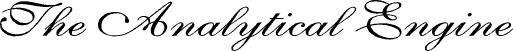Invented by Charles Babbage

### By L. F. MENABREAof Turin, Officer of the Military Engineers

from the Bibliothèque Universelle de Genève, October, 1842, No. 82

With notes upon the Memoir by the Translator
ADA AUGUSTA, COUNTESS OF LOVELACEThose labours which belong to the various branches of the mathematical sciences, although on first consideration they seem to be the exclusive province of intellect, may, nevertheless, be divided into two distinct sections; one of which may be called the mechanical, because it is subjected to precise and invariable laws, that are capable of being expressed by means of the operations of matter; while the other, demanding the intervention of reasoning, belongs more specially to the domain of the understanding. This admitted, we may propose to execute, by means of machinery, the mechanical branch of these labours, reserving for pure intellect that which depends on the reasoning faculties. Thus the rigid exactness of those laws which regulate numerical calculations must frequently have suggested the employment of material instruments, either for executing the whole of such calculations or for abridging them; and thence have arisen several inventions having this object in view, but which have in general but partially attained it. For instance, the much-admired machine of Pascal is now simply an object of curiosity, which, whilst it displays the powerful intellect of its inventor, is yet of little utility in itself. Its powers extended no further than the execution of the first four operations of arithmetic, and indeed were in reality confined to that of the first two, since multiplication and division were the result of a series of additions and subtractions. The chief drawback hitherto on most of such machines is, that they require the continual intervention of a human agent to regulate their movements, and thence arises a source of errors; so that, if their use has not become general for large numerical calculations, it is because they have not in fact resolved the double problem which the question presents, that of correctness in the results, united with economy of time.

Struck with similar reflections, Mr. Babbage has devoted some years to the realization of a gigantic idea. He proposed to himself nothing less than the construction of a machine capable of executing not merely arithmetical calculations, but even all those of analysis, if their laws are known. The imagination is at first astounded at the idea of such an undertaking; but the more calm reflection we bestow on it, the less impossible does success appear, and it is felt that it may depend on the discovery of some principle so general, that, if applied to machinery, the latter may be capable of mechanically translating the operations which may be indicated to it by algebraical notation. The illustrious inventor having been kind enough to communicate to me some of his views on this subject during a visit he made at Turin, I have, with his approbation, thrown together the impressions they have left on my mind. But the reader must not expect to find a description of Mr. Babbage's engine; the comprehension of this would entail studies of much length; and I shall endeavour merely to give an insight into the end proposed, and to develop the principles on which its attainment depends.

I must first premise that this engine is entirely different from that of which there is a notice in the ‘Treatise on the Economy of Machinery,’ by the same author. But as the latter gave rise to the idea of the engine in question, I consider it will be a useful preliminary briefly to recall what were Mr. Babbage's first essays, and also the circumstances in which they originated.

It is well known that the French government, wishing to promote the extension of the decimal system, had ordered the construction of logarithmical and trigonometrical tables of enormous extent. M. de Prony, who had been entrusted with the direction of this undertaking, divided it into three sections, to each of which was appointed a special class of persons. In the first section the formulæ were so combined as to render them subservient to the purposes of numerical calculation; in the second, these same formulæ were calculated for values of the variable, selected at certain successive distances; and under the third section, comprising about eighty individuals, who were most of them only acquainted with the first two rules of arithmetic, the values which were intermediate to those calculated by the second section were interpolated by means of simple additions and subtractions.

An undertaking similar to that just mentioned having been entered upon in England, Mr. Babbage conceived that the operations performed under the third section might be executed by a machine; and this idea he realized by means of mechanism, which has been in part put together, and to which the name Difference Engine is applicable, on account of the principle upon which its construction is founded. To give some notion of this, it will suffice to consider the series of whole square numbers, 1, 4, 9, 16, 25, 36, 49, 64, &c. By subtracting each of these from the succeeding one, we obtain a new series, which we will name the Series of First Differences, consisting of the numbers 3, 5, 7, 9, 11, 13, 15, &c. On subtracting from each of these the preceding one, we obtain the Second Differences, which are all constant and equal to 2. We may represent this succession of operations, and their results, in the following table.From the mode in which the last two columns B and C have been formed, it is easy to see, that if, for instance, we desire to pass from the number 5 to the succeeding one 7, we must add to the former the constant difference 2; similarly, if from the square number 9 we would pass to the following one 16, we must add to the former the difference 7, which difference is in other words the preceding difference 5, plus the constant difference 2; or again, which comes to the same thing, to obtain 16 we have only to add together the three numbers 2, 5, 9, placed obliquely in the direction ab. Similarly, we obtain the number 25 by summing up the three numbers placed in the oblique direction dc: commencing by the addition 2+7, we have the first difference 9 consecutively to 7; adding 16 to the 9 we have the square 25. We see then that the three numbers 2, 5, 9 being given, the whole series of successive square numbers, and that of their first differences likewise may be obtained by means of simple additions.

Now, to conceive how these operations may be reproduced by a machine, suppose the latter to have three dials, designated as A, B, C, on each of which are traced, say a thousand divisions, by way of example, over which a needle shall pass. The two dials, C, B, shall have in addition a registering hammer, which is to give a number of strokes equal to that of the divisions indicated by the needle. For each stroke of the registering hammer of the dial C, the needle B shall advance one division; similarly, the needle A shall advance one division for every stroke of the registering hammer of the dial B. Such is the general disposition of the mechanism.

This being understood, let us, at the beginning of the series of operations we wish to execute, place the needle C on the division 2, the needle B on the division 5, and the needle A on the division 9. Let us allow the hammer of the dial C to strike; it will strike twice, and at the same time the needle B will pass over two divisions. The latter will then indicate the number 7, which succeeds the number 5 in the column of first differences. If we now permit the hammer of the dial B to strike in its turn, it will strike seven times, during which the needle A will advance seven divisions; these added to the nine already marked by it will give the number 16, which is the square number consecutive to 9. If we now recommence these operations, beginning with the needle C, which is always to be left on the division 2, we shall perceive that by repeating them indefinitely, we may successively reproduce the series of whole square numbers by means of a very simple mechanism.

The theorem on which is based the construction of the machine we have just been describing, is a particular case of the following more general theorem: that if in any polynomial whatever, the highest power of whose variable is m, this same variable be increased by equal degrees; the corresponding values of the polynomial then calculated, and the first, second, third, &c. differences of these be taken (as for the preceding series of squares); the mth differences will all be equal to each other. So that, in order to reproduce the series of values of the polynomial by means of a machine analogous to the one above described, it is sufficient that there be (m+1) dials, having the mutual relations we have indicated. As the differences may be either positive or negative, the machine will have a contrivance for either advancing or retrograding each needle, according as the number to be algebraically added may have the sign plus or minus.

If from a polynomial we pass to a series having an infinite number of terms, arranged according to the ascending powers of the variable, it would at first appear, that in order to apply the machine to the calculation of the function represented by such a series, the mechanism must include an infinite number of dials, which would in fact render the thing impossible. But in many cases the difficulty will disappear, if we observe that for a great number of functions the series which represent them may be rendered convergent; so that, according to the degree of approximation desired, we may limit ourselves to the calculation of a certain number of terms of the series, neglecting the rest. By this method the question is reduced to the primitive case of a finite polynomial. It is thus that we can calculate the succession of the logarithms of numbers. But since, in this particular instance, the terms which had been originally neglected receive increments in a ratio so continually increasing for equal increments of the variable, that the degree of approximation required would ultimately be affected, it is necessary, at certain intervals, to calculate the value of the function by different methods, and then respectively to use the results thus obtained, as data whence to deduce, by means of the machine, the other intermediate values. We see that the machine here performs the office of the third section of calculators mentioned in describing the tables computed by order of the French government, and that the end originally proposed is thus fulfilled by it.

Such is the nature of the first machine which Mr. Babbage conceived. We see that its use is confined to cases where the numbers required are such as can be obtained by means of simple additions or subtractions; that the machine is, so to speak, merely the expression of one particular theorem of analysis; and that, in short, its operations cannot be extended so as to embrace the solution of an infinity of other questions included within the domain of mathematical analysis. It was while contemplating the vast field which yet remained to be traversed, that Mr. Babbage, renouncing his original essays, conceived the plan of another system of mechanism whose operations should themselves possess all the generality of algebraical notation, and which, on this account, he denominates the Analytical Engine.

Having now explained the state of the question, it is time for me to develop the principle on which is based the construction of this latter machine. When analysis is employed for the solution of any problem, there are usually two classes of operations to execute: first, the numerical calculation of the various coefficients; and secondly, their distribution in relation to the quantities affected by them. If, for example, we have to obtain the product of two binomials (a+bx) (m+nx), the result will be represented by am + (an + bm) x + bnx2, in which expression we must first calculate am, an, bm, bn; then take the sum of an + bm; and lastly, respectively distribute the coefficients thus obtained amongst the powers of the variable. In order to reproduce these operations by means of a machine, the latter must therefore possess two distinct sets of powers: first, that of executing numerical calculations; secondly, that of rightly distributing the values so obtained.

But if human intervention were necessary for directing each of these partial operations, nothing would be gained under the heads of correctness and economy of time; the machine must therefore have the additional requisite of executing by itself all the successive operations required for the solution of a problem proposed to it, when once the primitive numerical data for this same problem have been introduced. Therefore, since, from the moment that the nature of the calculation to be executed or of the problem to be resolved have been indicated to it, the machine is, by its own intrinsic power, of itself to go through all the intermediate operations which lead to the proposed result, it must exclude all methods of trial and guess-work, and can only admit the direct processes of calculation.

It is necessarily thus; for the machine is not a thinking being, but simply an automaton which acts according to the laws imposed upon it. This being fundamental, one of the earliest researches its author had to undertake, was that of finding means for effecting the division of one number by another without using the method of guessing indicated by the usual rules of arithmetic. The difficulties of effecting this combination were far from being among the least; but upon it depended the success of every other. Under the impossibility of my here explaining the process through which this end is attained, we must limit ourselves to admitting that the first four operations of arithmetic, that is addition, subtraction, multiplication and division, can be performed in a direct manner through the intervention of the machine. This granted, the machine is thence capable of performing every species of numerical calculation, for all such calculations ultimately resolve themselves into the four operations we have just named. To conceive how the machine can now go through its functions according to the laws laid down, we will begin by giving an idea of the manner in which it materially represents numbers.

Let us conceive a pile or vertical column consisting of an indefinite number of circular discs, all pierced through their centres by a common axis, around which each of them can take an independent rotatory movement. If round the edge of each of these discs are written the ten figures which constitute our numerical alphabet, we may then, by arranging a series of these figures in the same vertical line, express in this manner any number whatever. It is sufficient for this purpose that the first disc represent units, the second tens, the third hundreds, and so on. When two numbers have been thus written on two distinct columns, we may propose to combine them arithmetically with each other, and to obtain the result on a third column. In general, if we have a series of columns consisting of discs, which columns we will designate as V0, V1, V2, V3, V4, &c., we may require, for instance, to divide the number written on the column V1 by that on the column V4, and to obtain the result on the column V7. To effect this operation, we must impart to the machine two distinct arrangements; through the first it is prepared for executing a division, and through the second the columns it is to operate on are indicated to it, and also the column on which the result is to be represented. If this division is to be followed, for example, by the addition of two numbers taken on other columns, the two original arrangements of the machine must be simultaneously altered. If, on the contrary, a series of operations of the same nature is to be gone through, then the first of the original arrangements will remain, and the second alone must be altered Therefore, the arrangements that may be communicated to the various parts of the machine may be distinguished into two principal classes:

First, that relative to the Operations.
Secondly, that relative to the Variables.

By this latter we mean that which indicates the columns to be operated on. As for the operations themselves, they are executed by a special apparatus, which is designated by the name of mill, and which itself contains a certain number of columns, similar to those of the Variables. When two numbers are to be combined together, the machine commences by effacing them from the columns where they are written, that is, it places zero on every disc of the two vertical lines on which the numbers were represented; and it transfers the numbers to the mill. There, the apparatus having been disposed suitably for the required operation, this latter is effected, and, when completed, the result itself is transferred to the column of Variables which shall have been indicated. Thus the mill is that portion of the machine which works, and the columns of Variables constitute that where the results are represented and arranged. After the preceding explanations, we may perceive that all fractional and irrational results will be represented in decimal fractions. Supposing each column to have forty discs, this extension will be sufficient for all degrees of approximation generally required.

It will now be inquired how the machine can of itself, and without having recourse to the hand of man, assume the successive dispositions suited to the operations. The solution of this problem has been taken from Jacquard's apparatus, used for the manufacture of brocaded stuffs, in the following manner:—

Two species of threads are usually distinguished in woven stuffs; one is the warp or longitudinal thread, the other the woof or transverse thread, which is conveyed by the instrument called the shuttle, and which crosses the longitudinal thread or warp. When a brocaded stuff is required, it is necessary in turn to prevent certain threads from crossing the woof, and this according to a succession which is determined by the nature of the design that is to be reproduced. Formerly this process was lengthy and difficult, and it was requisite that the workman, by attending to the design which he was to copy, should himself regulate the movements the threads were to take. Thence arose the high price of this description of stuffs, especially if threads of various colours entered into the fabric. To simplify this manufacture, Jacquard devised the plan of connecting each group of threads that were to act together, with a distinct lever belonging exclusively to that group. All these levers terminate in rods, which are united together in one bundle, having usually the form of a parallelopiped with a rectangular base. The rods are cylindrical, and are separated from each other by small intervals. The process of raising the threads is thus resolved into that of moving these various lever-arms in the requisite order. To effect this, a rectangular sheet of pasteboard is taken, somewhat larger in size than a section of the bundle of lever-arms. If this sheet be applied to the base of the bundle, and an advancing motion be then communicated to the pasteboard, this latter will move with it all the rods of the bundle, and consequently the threads that are connected with each of them. But if the pasteboard, instead of being plain, were pierced with holes corresponding to the extremities of the levers which meet it, then, since each of the levers would pass through the pasteboard during the motion of the latter, they would all remain in their places. We thus see that it is easy so to determine the position of the holes in the pasteboard, that, at any given moment, there shall be a certain number of levers, and consequently of parcels of threads, raised, while the rest remain where they were. Supposing this process is successively repeated according to a law indicated by the pattern to be executed, we perceive that this pattern may be reproduced on the stuff. For this purpose we need merely compose a series of cards according to the law required, and arrange them in suitable order one after the other; then, by causing them to pass over a polygonal beam which is so connected as to turn a new face for every stroke of the shuttle, which face shall then be impelled parallelly to itself against the bundle of lever-arms, the operation of raising the threads will be regularly performed. Thus we see that brocaded tissues may be manufactured with a precision and rapidity formerly difficult to obtain.

Arrangements analogous to those just described have been introduced into the Analytical Engine. It contains two principal species of cards: first, Operation cards, by means of which the parts of the machine are so disposed as to execute any determinate series of operations, such as additions, subtractions, multiplications, and divisions; secondly, cards of the Variables, which indicate to the machine the columns on which the results are to be represented. The cards, when put in motion, successively arrange the various portions of the machine according to the nature of the processes that are to be effected, and the machine at the same time executes these processes by means of the various pieces of mechanism of which it is constituted.

In order more perfectly to conceive the thing, let us select as an example the resolution of two equations of the first degree with two unknown quantities. Let the following be the two equations, in which x and y are the unknown quantities:—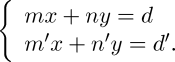We deduce, and for y an analogous expression. Let us continue to represent by V0, V1, V2, &c. the different columns which contain the numbers, and let us suppose that the first eight columns have been chosen for expressing on them the numbers represented by m, n, d, m', n', d', n and n', which implies that V0=m, V1=n, V2=d, V3=m', V4=n', V5=d', V6=n, V7=n'.

The series of operations commanded by the cards, and the results obtained, may be represented in the following table:—Since the cards do nothing but indicate in what manner and on what columns the machine shall act, it is clear that we must still, in every particular case, introduce the numerical data for the calculation. Thus, in the example we have selected, we must previously inscribe the numerical values of m, n, d, m', n', d', in the order and on the columns indicated, after which the machine when put in action will give the value of the unknown quantity x for this particular case. To obtain the value of y, another series of operations analogous to the preceding must be performed. But we see that they will be only four in number, since the denominator of the expression for y, excepting the sign, is the same as that for x, and equal to n'm-nm'. In the preceding table it will be remarked that the column for operations indicates four successive multiplications, two subtractions, and one division. Therefore, if desired, we need only use three operation-cards; to manage which, it is sufficient to introduce into the machine an apparatus which shall, after the first multiplication, for instance, retain the card which relates to this operation, and not allow it to advance so as to be replaced by another one, until after this same operation shall have been four times repeated. In the preceding example we have seen, that to find the value of x we must begin by writing the coefficients m, n, d, m', n', d', upon eight columns, thus repeating n and n' twice. According to the same method, if it were required to calculate y likewise, these coefficients must be written on twelve different columns. But it is possible to simplify this process, and thus to diminish the chances of errors, which chances are greater, the larger the number of the quantities that have to be inscribed previous to setting the machine in action. To understand this simplification, we must remember that every number written on a column must, in order to be arithmetically combined with another number, be effaced from the column on which it is, and transferred to the mill. Thus, in the example we have discussed, we will take the two coefficients m and n', which are each of them to enter into two different products, that is m into mn' and md', n' into mn' and n'd. These coefficients will be inscribed on the columns V0 and V4. If we commence the series of operations by the product of m into n', these numbers will be effaced from the columns V0 and V4, that they may be transferred to the mill, which will multiply them into each other, and will then command the machine to represent the result, say on the column V6. But as these numbers are each to be used again in another operation, they must again be inscribed somewhere; therefore, while the mill is working out their product, the machine will inscribe them anew on any two columns that may be indicated to it through the cards; and as, in the actual case, there is no reason why they should not resume their former places, we will suppose them again inscribed on V0 and V4, whence in short they would not finally disappear, to be reproduced no more, until they should have gone through all the combinations in which they might have to be used.

We see, then, that the whole assemblage of operations requisite for resolving the two above equations of the first degree may be definitely represented in the following table:—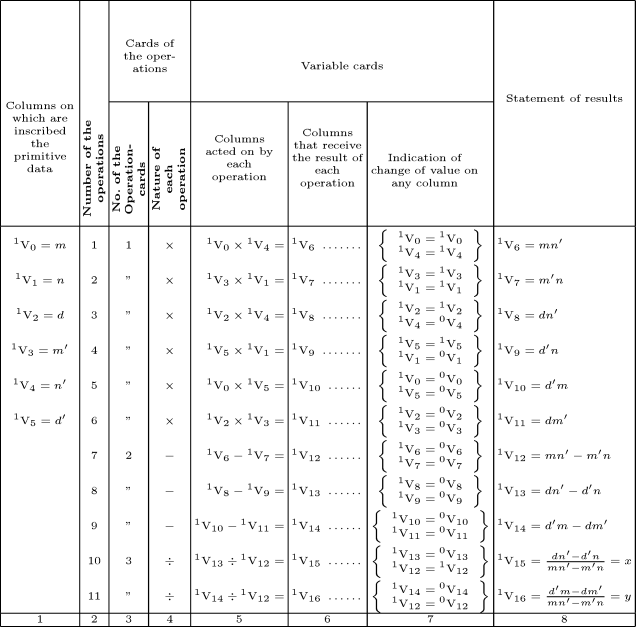Clicking on the table displays a larger image of it. Your browser's “Back” button will return you to this document.

In order to diminish to the utmost the chances of error in inscribing the numerical data of the problem, they are successively placed on one of the columns of the mill; then, by means of cards arranged for this purpose, these same numbers are caused to arrange themselves on the requisite columns, without the operator having to give his attention to it; so that his undivided mind may be applied to the simple inscription of these same numbers.

According to what has now been explained, we see that the collection of columns of Variables may be regarded as a store of numbers, accumulated there by the mill, and which, obeying the orders transmitted to the machine by means of the cards, pass alternately from the mill to the store and from the store to the mill, that they may undergo the transformations demanded by the nature of the calculation to be performed.

Hitherto no mention has been made of the signs in the results, and the machine would be far from perfect were it incapable of expressing and combining amongst each other positive and negative quantities. To accomplish this end, there is, above every column, both of the mill and of the store, a disc, similar to the discs of which the columns themselves consist. According as the digit on this disc is even or uneven, the number inscribed on the corresponding column below it will be considered as positive or negative. This granted, we may, in the following manner, conceive how the signs can be algebraically combined in the machine. When a number is to be transferred from the store to the mill, and vice versâ, it will always be transferred with its sign, which will effected by means of the cards, as has been explained in what precedes. Let any two numbers then, on which we are to operate arithmetically, be placed in the mill with their respective signs. Suppose that we are first to add them together; the operation-cards will command the addition: if the two numbers be of the same sign, one of the two will be entirely effaced from where it was inscribed, and will go to add itself on the column which contains the other number; the machine will, during this operation, be able, by means of a certain apparatus, to prevent any movement in the disc of signs which belongs to the column on which the addition is made, and thus the result will remain with the sign which the two given numbers originally had. When two numbers have two different signs, the addition commanded by the card will be changed into a subtraction through the intervention of mechanisms which are brought into play by this very difference of sign. Since the subtraction can only be effected on the larger of the two numbers, it must be arranged that the disc of signs of the larger number shall not move while the smaller of the two numbers is being effaced from its column and subtracted from the other, whence the result will have the sign of this latter, just as in fact it ought to be. The combinations to which algebraical subtraction give rise, are analogous to the preceding. Let us pass on to multiplication. When two numbers to be multiplied are of the same sign, the result is positive; if the signs are different, the product must be negative. In order that the machine may act conformably to this law, we have but to conceive that on the column containing the product of the two given numbers, the digit which indicates the sign of that product has been formed by the mutual addition of the two digits that respectively indicated the signs of the two given numbers; it is then obvious that if the digits of the signs are both even, or both odd, their sum will be an even number, and consequently will express a positive number; but that if, on the contrary, the two digits of the signs are one even and the other odd, their sum will be an odd number, and will consequently express a negative number. In the case of division. instead of adding the digits of the discs, they must be subtracted one from the other, which will produce results analogous to the preceding; that is to say, that if these figures are both even or both uneven, the remainder of this subtraction will be even; and it will be uneven in the contrary case. When I speak of mutually adding or subtracting the numbers expressed by the digits of the signs, I merely mean that one of the sign-discs is made to advance or retrograde a number of divisions equal to that which is expressed by the digit on the other sign-disc. We see, then, from the preceding explanation, that it is possible mechanically to combine the signs of quantities so as to obtain results conformable to those indicated by algebra.

The machine is not only capable of executing those numerical calculations which depend on a given algebraical formula, but it is also fitted for analytical calculations in which there are one or several variables to be considered. It must be assumed that the analytical expression to be operated on can be developed according to powers of the variable, or according to determinate functions of this same variable, such as circular functions, for instance; and similarly for the result that is to be attained. If we then suppose that above the columns of the store, we have inscribed the powers or the functions of the variable, arranged according to whatever is the prescribed law of development, the coefficients of these several terms may be respectively placed on the corresponding column below each. In this manner we shall have a representation of an analytical development; and, supposing the position of the several terms composing it to be invariable, the problem will be reduced to that of calculating their coefficients according to the laws demanded by the nature of the question. In order to make this more clear, we shall take the following very simple example, in which we are to multiply (a + bx1) by (A + B cos1 x). We shall begin by writing x0 , x1, cos0 x, cos1 x, above the columns V0, V1, V2, V3; then since, from the form of the two functions to be combined, the terms which are to compose the products will be of the following nature, x0·cos0 x, x0·cos1 x, x1·cos0 x, x1·cos1 x, these will be inscribed above the columns V4, V5, V6, V7. The coefficients of x0, x1, cos0 x, cos1 x being given, they will, by means of the mill, be passed to the columns V0, V1, V2 and V3. Such are the primitive data of the problem. It is now the business of the machine to work out its solution, that is, to find the coefficients which are to be inscribed on V4, V5, V6, V7. To attain this object, the law of formation of these same coefficients being known, the machine will act through the intervention of the cards, in the manner indicated by the following table:—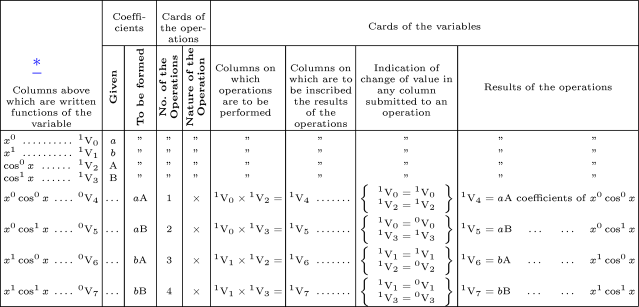Clicking on the table displays a larger image of it. Your browser's “Back” button will return you to this document. Read footnote in diagram.

It will now be perceived that a general application may be made of the principle developed in the preceding example, to every species of process which it may be proposed to effect on series submitted to calculation. It is sufficient that the law of formation of the coefficients be known, and that this law be inscribed on the cards of the machine, which will then of itself execute all the calculations requisite for arriving at the proposed result. If, for instance, a recurring series were proposed, the law of formation of the coefficients being here uniform, the same operations which must be performed for one of them will be repeated for all the others; there will merely be a change in the locality of the operation, that is, it will be performed with different columns. Generally, since every analytical expression is susceptible of being expressed in a series ordered according to certain functions of the variable, we perceive that the machine will include all analytical calculations which can be definitively reduced to the formation of coefficients according to certain laws, and to the distribution of these with respect to the variables.

We may deduce the following important consequence from these explanations, viz. that since the cards only indicate the nature of the operations to be performed, and the columns of Variables with which they are to be executed, these cards will themselves possess all the generality of analysis, of which they are in fact merely a translation. We shall now further examine some of the difficulties which the machine must surmount, if its assimilation to analysis is to be complete. There are certain functions which necessarily change in nature when they pass through zero or infinity, or whose values cannot be admitted when they pass these limits. When such cases present themselves, the machine is able, by means of a bell, to give notice that the passage through zero or infinity is taking place, and it then stops until the attendant has again set it in action for whatever process it may next be desired that it shall perform. If this process has been foreseen, then the machine, instead of ringing, will so dispose itself as to present the new cards which have relation to the operation that is to succeed the passage through zero and infinity. These new cards may follow the first, but may only come into play contingently upon one or other of the two circumstances just mentioned taking place.

Let us consider a term of the form abn; since the cards are but a translation of the analytical formula, their number in this particular case must be the same, whatever be the value of n; that is to say, whatever be the number of multiplications required for elevating b to the nth power (we are supposing for the moment that n is a whole number). Now, since the exponent n indicates that b is to be multiplied n times by itself, and all these operations are of the same nature, it will be sufficient to employ one single operation-card, viz. that which orders the multiplication.

But when n is given for the particular case to be calculated, it will be further requisite that the machine limit the number of its multiplications according to the given values. The process may be thus arranged. The three numbers a, b and n will be written on as many distinct columns of the store; we shall designate them V0, V1, V2; the result abn will place itself on the column V3. When the number n has been introduced into the machine, a card will order a certain registering-apparatus to mark (n-1), and will at the same time execute the multiplication of b by b. When this is completed, it will be found that the registering-apparatus has effaced a unit, and that it only marks (n−2); while the machine will now again order the number b written on the column V1 to multiply itself with the product b2 written on the column V3, which will give b3. Another unit is then effaced from the registering-apparatus, and the same processes are continually repeated until it only marks zero. Thus the number bn will be found inscribed on V3, when the machine, pursuing its course of operations, will order the product of bn by a; and the required calculation will have been completed without there being any necessity that the number of operation-cards used should vary with the value of n. If n were negative, the cards, instead of ordering the multiplication of a by bn, would order its division; this we can easily conceive, since every number, being inscribed with its respective sign, is consequently capable of reacting on the nature of the operations to be executed. Finally, if n were fractional, of the form p/q, an additional column would be used for the inscription of q, and the machine would bring into action two sets of processes, one for raising b to the power p, the other for extracting the qth root of the number so obtained.

Again, it may be required, for example, to multiply an expression of the form axm+bxn by another Axp+Bxq, and then to reduce the product to the least number of terms, if any of the indices are equal. The two factors being ordered with respect to x, the general result of the multiplication would be Aaxm+p+Abxn+p+Baxm+q+Bbxn+q. Up to this point the process presents no difficulties; but suppose that we have m=p and n=q, and that we wish to reduce the two middle terms to a single one (Ab+Ba)xm+q. For this purpose, the cards may order m+q and n+p to be transferred into the mill, and there subtracted one from the other; if the remainder is nothing, as would be the case on the present hypothesis, the mill will order other cards to bring to it the coefficients Ab and Ba, that it may add them together and give them in this state as a coefficient for the single term xn+p=xm+q.

This example illustrates how the cards are able to reproduce all the operations which intellect performs in order to attain a determinate result, if these operations are themselves capable of being precisely defined.

Let us now examine the following expression:—which we know becomes equal to the ratio of the circumference to the diameter, when n is infinite. We may require the machine not only to perform the calculation of this fractional expression, but further to give indication as soon as the value becomes identical with that of the ratio of the circumference to the diameter when n is infinite, a case in which the computation would be impossible. Observe that we should thus require of the machine to interpret a result not of itself evident, and that this is not amongst its attributes, since it is no thinking being. Nevertheless, when the cos of n=1/0 has been foreseen, a card may immediately order the substitution of the value of π (π being the ratio of the circumference to the diameter), without going through the series of calculations indicated. This would merely require that the machine contain a special card, whose office it should be to place the number π in a direct and independent manner on the column indicated to it. And here we should introduce the mention of a third species of cards, which may be called cards of numbers. There are certain numbers, such as those expressing the ratio of the circumference to the diameter, the Numbers of Bernoulli, &c., which frequently present themselves in calculations. To avoid the necessity for computing them every time they have to be used, certain cards may be combined specially in order to give these numbers ready made into the mill, whence they afterwards go and place themselves on those columns of the store that are destined for them. Through this means the machine will be susceptible of those simplifications afforded by the use of numerical tables. It would be equally possible to introduce, by means of these cards, the logarithms of numbers; but perhaps it might not be in this case either the shortest or the most appropriate method; for the machine might be able to perform the same calculations by other more expeditious combinations, founded on the rapidity with which it executes the first four operations of arithmetic. To give an idea of this rapidity, we need only mention that Mr. Babbage believes he can, by his engine, form the product of two numbers, each containing twenty figures, in three minutes.

Perhaps the immense number of cards required for the solution of any rather complicated problem may appear to be an obstacle; but this does not seem to be the case. There is no limit to the number of cards that can be used. Certain stuffs require for their fabrication not less than twenty thousand cards, and we may unquestionably far exceed even this quantity.

Resuming what we have explained concerning the Analytical Engine, we may conclude that it is based on two principles: the first consisting in the fact that every arithmetical calculation ultimately depends on four principal operations—addition, subtraction, multiplication, and division; the second, in the possibility of reducing every analytical calculation to that of the coefficients for the several terms of a series. If this last principle be true, all the operations of analysis come within the domain of the engine. To take another point of view: the use of the cards offers a generality equal to that of algebraical formulæ, since such a formula simply indicates the nature and order of the operations requisite for arriving at a certain definite result, and similarly the cards merely command the engine to perform these same operations; but in order that the mechanisms may be able to act to any purpose, the numerical data of the problem must in every particular case be introduced. Thus the same series of cards will serve for all questions whose sameness of nature is such as to require nothing altered excepting the numerical data. In this light the cards are merely a translation of algebraical formulæ, or, to express it better, another form of analytical notation.

Since the engine has a mode of acting peculiar to itself, it will in every particular case be necessary to arrange the series of calculations conformably to the means which the machine possesses; for such or such a process which might be very easy for a calculator may be long and complicated for the engine, and vice versâ.

Considered under the most general point of view, the essential object of the machine being to calculate, according to the laws dictated to it, the values of numerical coefficients which it is then to distribute appropriately on the columns which represent the variables, it follows that the interpretation of formulæ and of results is beyond its province, unless indeed this very interpretation be itself susceptible of expression by means of the symbols which the machine employs. Thus, although it is not itself the being that reflects, it may yet be considered as the being which executes the conceptions of intelligence. The cards receive the impress of these conceptions, and transmit to the various trains of mechanism composing the engine the orders necessary for their action. When once the engine shall have been constructed, the difficulty will be reduced to the making out of the cards; but as these are merely the translation of algebraical formulæ, it will, by means of some simple notations, be easy to consign the execution of them to a workman. Thus the whole intellectual labour will be limited to the preparation of the formulæ, which must be adapted for calculation by the engine.

Now, admitting that such an engine can be constructed, it may be inquired: what will be its utility? To recapitulate; it will afford the following advantages:—First, rigid accuracy. We know that numerical calculations are generally the stumbling-block to the solution of problems, since errors easily creep into them, and it is by no means always easy to detect these errors. Now the engine, by the very nature of its mode of acting, which requires no human intervention during the course of its operations, presents every species of security under the head of correctness: besides, it carries with it its own check; for at the end of every operation it prints off, not only the results, but likewise the numerical data of the question; so that it is easy to verify whether the question has been correctly proposed. Secondly, economy of time: to convince ourselves of this, we need only recollect that the multiplication of two numbers, consisting each of twenty figures, requires at the very utmost three minutes. Likewise, when a long series of identical computations is to be performed, such as those required for the formation of numerical tables, the machine can be brought into play so as to give several results at the same time, which will greatly abridge the whole amount of the processes. Thirdly, economy of intelligence: a simple arithmetical computation requires to be performed by a person possessing some capacity; and when we pass to more complicated calculations, and wish to use algebraical formulæ in particular cases, knowledge must be possessed which presupposes preliminary mathematical studies of some extent. Now the engine, from its capability of performing by itself all these purely material operations, spares intellectual labour, which may be more profitably employed. Thus the engine may be considered as a real manufactory of figures, which will lend its aid to those many useful sciences and arts that depend on numbers. Again, who can foresee the consequences of such an invention? In truth, how many precious observations remain practically barren for the progress of the sciences, because there are not powers sufficient for computing the results! And what discouragement does the perspective of a long and arid computation cast into the mind of a man of genius, who demands time exclusively for meditation, and who beholds it snatched from him by the material routine of operations! Yet it is by the laborious route of analysis that he must reach truth; but he cannot pursue this unless guided by numbers; for without numbers it is not given us to raise the veil which envelopes the mysteries of nature. Thus the idea of constructing an apparatus capable of aiding human weakness in such researches, is a conception which, being realized, would mark a glorious epoch in the history of the sciences. The plans have been arranged for all the various parts, and for all the wheel-work, which compose this immense apparatus, and their action studied; but these have not yet been fully combined together in the drawings and mechanical notation. The confidence which the genius of Mr. Babbage must inspire, affords legitimate ground for hope that this enterprise will be crowned with success; and while we render homage to the intelligence which directs it, let us breathe aspirations for the accomplishment of such an undertaking.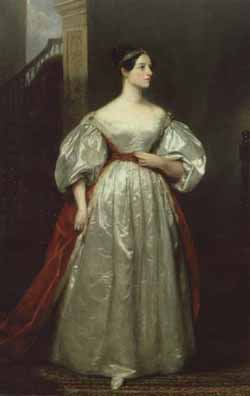Ada Augusta,Countess of Lovelace

## NOTES BY THE TRANSLATOR

### Note A

The particular function whose integral the Difference Engine was constructed to tabulate, is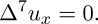The purpose which that engine has been specially intended and adapted to fulfil, is the computation of nautical and astronomical tables. The integral of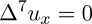being uz = a+bx+cx2+dx3+ex4+fx5+gx6,

the constants a, b, c, &c. are represented on the seven columns of discs, of which the engine consists. It can therefore tabulate accurately and to an unlimited extent, all series whose general term is comprised in the above formula; and it can also tabulate approximatively between intervals of greater or less extent, all other series which are capable of tabulation by the Method of Differences.

The Analytical Engine, on the contrary, is not merely adapted for tabulating the results of one particular function and of no other, but for developing and tabulating any function whatever. In fact the engine may be described as being the material expression of any indefinite function of any degree of generality and complexity, such as for instance,

F(x, y, z, log x, sin y, x p, &c.),

which is, it will be observed, a function of all other possible functions of any number of quantities.

In this, which we may call the neutral or zero state of the engine, it is ready to receive at any moment, by means of cards constituting a portion of its mechanism (and applied on the principle of those used in the Jacquard-loom), the impress of whatever special function we may desire to develope or to tabulate. These cards contain within themselves (in a manner explained in the Memoir itself) the law of development of the particular function that may be under consideration, and they compel the mechanism to act accordingly in a certain corresponding order. One of the simplest cases would be for example, to suppose that

F(x, y, z, &c. &c.)

is the particular function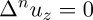which the Difference Engine tabulates for values of n only up to 7. In this case the cards would order the mechanism to go through that succession of operations which would tabulate

uz = a + bx + cx2 + ··· + mxn−1

where n might be any number whatever.

These cards, however, have nothing to do with the regulation of the particular numerical data. They merely determine the operations to be effected, which operations may of course be performed on an infinite variety of particular numerical values, and do not bring out any definite numerical results unless the numerical data of the problem have been impressed on the requisite portions of the train of mechanism. In the above example, the first essential step towards an arithmetical result would be the substitution of specific numbers for n, and for the other primitive quantities which enter into the function.

Again, let us suppose that for F we put two complete equations of the fourth degree between x and y. We must then express on the cards the law of elimination for such equations. The engine would follow out those laws, and would ultimately give the equation of one variable which results from such elimination. Various modes of elimination might be selected; and of course the cards must be made out accordingly. The following is one mode that might be adopted. The engine is able to multiply together any two functions of the form

a + bx + cx2 + ··· + pxn.

This granted, the two equations may be arranged according to the powers of y, and the coefficients of the powers of y may be arranged according to powers of x. The elimination of y will result from the successive multiplications and subtractions of several such functions. In this, and in all other instances, as was explained above, the particular numerical data and the numerical results are determined by means and by portions of the mechanism which act quite independently of those that regulate the operations.

In studying the action of the Analytical Engine, we find that the peculiar and independent nature of the considerations which in all mathematical analysis belong to operations, as distinguished from the objects operated upon and from the results of the operations performed upon those objects, is very strikingly defined and separated.

It is well to draw attention to this point, not only because its full appreciation is essential to the attainment of any very just and adequate general comprehension of the powers and mode of action of the Analytical Engine, but also because it is one which is perhaps too little kept in view in the study of mathematical science in general. It is, however, impossible to confound it with other considerations, either when we trace the manner in which that engine attains its results, or when we prepare the data for its attainment of those results. It were much to be desired, that when mathematical processes pass through the human brain instead of through the medium of inanimate mechanism, it were equally a necessity of things that the reasonings connected with operations should hold the same just place as a clear and well-defined branch of the subject of analysis, a fundamental but yet independent ingredient in the science, which they must do in studying the engine. The confusion, the difficulties, the contradictions which, in consequence of a want of accurate distinctions in this particular, have up to even a recent period encumbered mathematics in all those branches involving the consideration of negative and impossible quantities, will at once occur to the reader who is at all versed in this science, and would alone suffice to justify dwelling somewhat on the point, in connexion with any subject so peculiarly fitted to give forcible illustration of it as the Analytical Engine. It may be desirable to explain, that by the word operation, we mean any process which alters the mutual relation of two or more things, be this relation of what kind it may. This is the most general definition, and would include all subjects in the universe. In abstract mathematics, of course operations alter those particular relations which are involved in the considerations of number and space, and the results of operations are those peculiar results which correspond to the nature of the subjects of operation. But the science of operations, as derived from mathematics more especially, is a science of itself, and has its own abstract truth and value; just as logic has its own peculiar truth and value, independently of the subjects to which we may apply its reasonings and processes. Those who are accustomed to some of the more modern views of the above subject, will know that a few fundamental relations being true, certain other combinations of relations must of necessity follow; combinations unlimited in variety and extent if the deductions from the primary relations be carried on far enough. They will also be aware that one main reason why the separate nature of the science of operations has been little felt, and in general little dwelt on, is the shifting meaning of many of the symbols used in mathematical notation. First, the symbols of operation are frequently also the symbols of the results of operations. We may say that these symbols are apt to have both a retrospective and a prospective signification. They may signify either relations that are the consequences of a series of processes already performed, or relations that are yet to be effected through certain processes. Secondly, figures, the symbols of numerical magnitude, are frequently also the symbols of operations, as when they are the indices of powers. Wherever terms have a shifting meaning, independent sets of considerations are liable to become complicated together, and reasonings and results are frequently falsified. Now in the Analytical Engine, the operations which come under the first of the above heads are ordered and combined by means of a notation and of a train of mechanism which belong exclusively to themselves; and with respect to the second head, whenever numbers meaning operations and not quantities (such as the indices of powers) are inscribed on any column or set of columns, those columns immediately act in a wholly separate and independent manner, becoming connected with the operating mechanism exclusively, and re-acting upon this. They never come into combination with numbers upon any other columns meaning quantities; though, of course, if there are numbers meaning operations upon n columns, these may combine amongst each other, and will often be required to do so, just as numbers meaning quantities combine with each other in any variety. It might have been arranged that all numbers meaning operations should have appeared on some separate portion of the engine from that which presents numerical quantities; but the present mode is in some cases more simple, and offers in reality quite as much distinctness when understood.

The operating mechanism can even be thrown into action independently of any object to operate upon (although of course no result could then be developed). Again, it might act upon other things besides number, were objects found whose mutual fundamental relations could be expressed by those of the abstract science of operations, and which should be also susceptible of adaptations to the action of the operating notation and mechanism of the engine. Supposing, for instance, that the fundamental relations of pitched sounds in the science of harmony and of musical composition were susceptible of such expression and adaptations, the engine might compose elaborate and scientific pieces of music of any degree of complexity or extent.

The Analytical Engine is an embodying of the science of operations, constructed with peculiar reference to abstract number as the subject of those operations. The Difference Engine is the embodying of one particular and very limited set of operations, which (see the notation used in Note B) may be expressed thus (+, +, +, +, +, +), or thus, 6(+). Six repetitions of the one operation, +, is, in fact, the whole sum and object of that engine. It has seven columns, and a number on any column can add itself to a number on the next column to its right-hand. So that, beginning with the column furthest to the left, six additions can be effected, and the result appears on the seventh column, which is the last on the right-hand. The operating mechanism of this engine acts in as separate and independent a manner as that of the Analytical Engine; but being susceptible of only one unvarying and restricted combination, it has little force or interest in illustration of the distinct nature of the science of operations. The importance of regarding the Analytical Engine under this point of view will, we think, become more and more obvious as the reader proceeds with M. Menabrea's clear and masterly article. The calculus of operations is likewise in itself a topic of so much interest, and has of late years been so much more written on and thought on than formerly, that any bearing which that engine, from its mode of constitution, may possess upon the illustration of this branch of mathematical science should not be overlooked. Whether the inventor of this engine had any such views in his mind while working out the invention, or whether he may subsequently ever have regarded it under this phase, we do not know; but it is one that forcibly occurred to ourselves on becoming acquainted with the means through which analytical combinations are actually attained by the mechanism. We cannot forbear suggesting one practical result which it appears to us must be greatly facilitated by the independent manner in which the engine orders and combines its operations: we allude to the attainment of those combinations into which imaginary quantities enter. This is a branch of its processes into which we have not had the opportunity of inquiring, and our conjecture therefore as to the principle on which we conceive the accomplishment of such results may have been made to depend, is very probably not in accordance with the fact, and less subservient for the purpose than some other principles, or at least requiring the cooperation of others. It seems to us obvious, however, that where operations are so independent in their mode of acting, it must be easy, by means of a few simple provisions, and additions in arranging the mechanism, to bring out a double set of results, viz.—1st, the numerical magnitudes which are the results of operations performed on numerical data. (These results are the primary object of the engine.) 2ndly, the symbolical results to be attached to those numerical results, which symbolical results are not less the necessary and logical consequences of operations performed upon symbolical data, than are numerical results when the data are numerical.

If we compare together the powers and the principles of construction of the Difference and of the Analytical Engines, we shall perceive that the capabilities of the latter are immeasurably more extensive than those of the former, and that they in fact hold to each other the same relationship as that of analysis to arithmetic. The Difference Engine can effect but one particular series of operations, viz. that required for tabulating the integral of the special function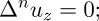and as it can only do this for values of n up to 7, it cannot be considered as being the most general expression even of one particular function, much less as being the expression of any and all possible functions of all degrees of generality. The Difference Engine can in reality (as has been already partly explained) do nothing but add; and any other processes, not excepting those of simple subtraction, multiplication and division, can be performed by it only just to that extent in which it is possible, by judicious mathematical arrangement and artifices, to reduce them to a series of additions. The method of differences is, in fact, a method of additions; and as it includes within its means a larger number of results attainable by addition simply, than any other mathematical principle, it was very appropriately selected as the basis on which to construct an Adding Machine, so as to give to the powers of such a machine the widest possible range. The Analytical Engine, on the contrary, can either add, subtract, multiply or divide with equal facility; and performs each of these four operations in a direct manner, without the aid of any of the other three. This one fact implies everything; and it is scarcely necessary to point out, for instance, that while the Difference Engine can merely tabulate, and is incapable of developing, the Analytical Engine can either tabulate or develope.

The former engine is in its nature strictly arithmetical, and the results it can arrive at lie within a very clearly defined and restricted range, while there is no finite line of demarcation which limits the powers of the Analytical Engine. These powers are co-extensive with our knowledge of the laws of analysis itself, and need be bounded only by our acquaintance with the latter. Indeed we may consider the engine as the material and mechanical representative of analysis, and that our actual working powers in this department of human study will be enabled more effectually than heretofore to keep pace with our theoretical knowledge of its principles and laws, through the complete control which the engine gives us over the executive manipulation of algebraical and numerical symbols.

Those who view mathematical science, not merely as a vast body of abstract and immutable truths, whose intrinsic beauty, symmetry and logical completeness, when regarded in their connexion together as a whole, entitle them to a prominent place in the interest of all profound and logical minds, but as possessing a yet deeper interest for the human race, when it is remembered that this science constitutes the language through which alone we can adequately express the great facts of the natural world, and those unceasing changes of mutual relationship which, visibly or invisibly, consciously or unconsciously to our immediate physical perceptions, are interminably going on in the agencies of the creation we live amidst: those who thus think on mathematical truth as the instrument through which the weak mind of man can most effectually read his Creator's works, will regard with especial interest all that can tend to facilitate the translation of its principles into explicit practical forms.

The distinctive characteristic of the Analytical Engine, and that which has rendered it possible to endow mechanism with such extensive faculties as bid fair to make this engine the executive right-hand of abstract algebra, is the introduction into it of the principle which Jacquard devised for regulating, by means of punched cards, the most complicated patterns in the fabrication of brocaded stuffs. It is in this that the distinction between the two engines lies. Nothing of the sort exists in the Difference Engine. We may say most aptly, that the Analytical Engine weaves algebraical patterns just as the Jacquard-loom weaves flowers and leaves. Here, it seems to us, resides much more of originality than the Difference Engine can be fairly entitled to claim. We do not wish to deny to this latter all such claims. We believe that it is the only proposal or attempt ever made to construct a calculating machine founded on the principle of successive orders of differences, and capable of printing off its own results; and that this engine surpasses its predecessors, both in the extent of the calculations which it can perform, in the facility, certainty and accuracy with which it can effect them, and in the absence of all necessity for the intervention of human intelligence during the performance of its calculations. Its nature is, however, limited to the strictly arithmetical, and it is far from being the first or only scheme for constructing arithmetical calculating machines with more or less of success.

The bounds of arithmetic were however outstepped the moment the idea of applying the cards had occurred; and the Analytical Engine does not occupy common ground with mere “calculating machines.” It holds a position wholly its own; and the considerations it suggests are most interesting in their nature. In enabling mechanism to combine together general symbols in successions of unlimited variety and extent, a uniting link is established between the operations of matter and the abstract mental processes of the most abstract branch of mathematical science. A new, a vast, and a powerful language is developed for the future use of analysis, in which to wield its truths so that these may become of more speedy and accurate practical application for the purposes of mankind than the means hitherto in our possession have rendered possible. Thus not only the mental and the material, but the theoretical and the practical in the mathematical world, are brought into more intimate and effective connexion with each other. We are not aware of its being on record that anything partaking in the nature of what is so well designated the Analytical Engine has been hitherto proposed, or even thought of, as a practical possibility, any more than the idea of a thinking or of a reasoning machine.

We will touch on another point which constitutes an important distinction in the modes of operating of the Difference and Analytical Engines. In order to enable the former to do its business, it is necessary to put into its columns the series of numbers constituting the first terms of the several orders of differences for whatever is the particular table under consideration. The machine then works upon these as its data. But these data must themselves have been already computed through a series of calculations by a human head. Therefore that engine can only produce results depending on data which have been arrived at by the explicit and actual working out of processes that are in their nature different from any that come within the sphere of its own powers. In other words, an analysing process must have been gone through by a human mind in order to obtain the data upon which the engine then synthetically builds its results. The Difference Engine is in its character exclusively synthetical, while the Analytical Engine is equally capable of analysis or of synthesis.

It is true that the Difference Engine can calculate to a much greater extent with these few preliminary data, than the data themselves required for their own determination. The table of squares, for instance, can be calculated to any extent whatever, when the numbers one and two are furnished; and a very few differences computed at any part of a table of logarithms would enable the engine to calculate many hundreds or even thousands of logarithms. Still the circumstance of its requiring, as a previous condition, that any function whatever shall have been numerically worked out, makes it very inferior in its nature and advantages to an engine which, like the Analytical Engine, requires merely that we should know the succession and distribution of the operations to be performed; without there being any occasion, in order to obtain data on which it can work, for our ever having gone through either the same particular operations which it is itself to effect, or any others. Numerical data must of course be given it, but they are mere arbitrary ones; not data that could only be arrived at through a systematic and necessary series of previous numerical calculations, which is quite a different thing.

To this it may be replied, that an analysing process must equally have been performed in order to furnish the Analytical Engine with the necessary operative data; and that herein may also lie a possible source of error. Granted that the actual mechanism is unerring in its processes, the cards may give it wrong orders. This is unquestionably the case; but there is much less chance of error, and likewise far less expenditure of time and labour, where operations only, and the distribution of these operations, have to be made out, than where explicit numerical results are to be attained. In the case of the Analytical Engine we have undoubtedly to lay out a certain capital of analytical labour in one particular line; but this is in order that the engine may bring us in a much larger return in another line. It should be remembered also that the cards, when once made out for any formula, have all the generality of algebra, and include an infinite number of particular cases.

We have dwelt considerably on the distinctive peculiarities of each of these engines, because we think it essential to place their respective attributes in strong relief before the apprehension of the public; and to define with clearness and accuracy the wholly different nature of the principles on which each is based, so as to make it self-evident to the reader (the mathematical reader at least) in what manner and degree the powers of the Analytical Engine transcend those of an engine, which, like the Difference Engine, can only work out such results as may be derived from one restricted and particular series of processes, such as those included in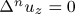. We think this of importance, because we know that there exists considerable vagueness and inaccuracy in the mind of persons in general on the subject. There is a misty notion amongst most of those who have attended at all to it, that two “calculating machines” have been successively invented by the same person within the last few years; while others again have never heard but of the one original “calculating machine,” and are not aware of there being any extension upon this. For either of these two classes of persons the above considerations are appropriate. While the latter require a knowledge of the fact that there are two such inventions, the former are not less in want of accurate and well-defined information on the subject. No very clear or correct ideas prevail as to the characteristics of each engine, or their respective advantages or disadvantages; and in meeting with those incidental allusions, of a more or less direct kind, which occur in so many publications of the day, to these machines, it must frequently be matter of doubt which “calculating machine” is referred to, or whether both are included in the general allusion.

We are desirous likewise of removing two misapprehensions which we know obtain, to some extent, respecting these engines. In the first place it is very generally supposed that the Difference Engine, after it had been completed up to a certain point, suggested the idea of the Analytical Engine; and that the second is in fact the improved offspring of the first, and grew out of the existence of its predecessor, through some natural or else accidental combination of ideas suggested by this one. Such a supposition is in this instance contrary to the facts; although it seems to be almost an obvious inference, wherever two inventions, similar in their nature and objects, succeed each other closely in order of time, and strikingly in order of value; more especially when the same individual is the author of both. Nevertheless the ideas which led to the Analytical Engine occurred in a manner wholly independent of any that were connected with the Difference Engine. These ideas are indeed in their own intrinsic nature independent of the latter engine, and might equally have occurred had it never existed nor been even thought of at all.

The second of the misapprehensions above alluded to relates to the well-known suspension, during some years past, of all progress in the construction of the Difference Engine. Respecting the circumstances which have interfered with the actual completion of either invention, we offer no opinion; and in fact are not possessed of the data for doing so, had we the inclination. But we know that some persons suppose these obstacles (be they what they may) to have arisen in consequence of the subsequent invention of the Analytical Engine while the former was in progress. We have ourselves heard it even lamented that an idea should ever have occurred at all, which had turned out to be merely the means of arresting what was already in a course of successful execution, without substituting the superior invention in its stead. This notion we can contradict in the most unqualified manner. The progress of the Difference Engine had long been suspended, before there were even the least crude glimmerings of any invention superior to it. Such glimmerings, therefore, and their subsequent development, were in no way the original cause of that suspension; although, where difficulties of some kind or other evidently already existed, it was not perhaps calculated to remove or lessen them that an invention should have been meanwhile thought of, which, while including all that the first was capable of, possesses powers so extended as to eclipse it altogether.

We leave it for the decision of each individual (after he has possessed himself of competent information as to the characteristics of each engine) to determine how far it ought to be matter of regret that such an accession has been made to the powers of human science, even if it has (which we greatly doubt) increased to a certain limited extent some already existing difficulties that had arisen in the way of completing a valuable but lesser work. We leave it for each to satisfy himself as to the wisdom of desiring the obliteration (were that now possible) of all records of the more perfect invention, in order that the comparatively limited one might be finished. The Difference Engine would doubtless fulfil all those practical objects which it was originally destined for. It would certainly calculate all the tables that are more directly necessary for the physical purposes of life, such as nautical and other computations. Those who incline to very strictly utilitarian views may perhaps feel that the peculiar powers of the Analytical Engine bear upon questions of abstract and speculative science, rather than upon those involving every-day and ordinary human interests. These persons being likely to possess but little sympathy, or possibly acquaintance, with any branches of science which they do not find to be useful (according to their definition of that word), may conceive that the undertaking of that engine, now that the other one is already in progress, would be a barren and unproductive laying out of yet more money and labour; in fact, a work of supererogation. Even in the utilitarian aspect, however, we do not doubt that very valuable practical results would be developed by the extended faculties of the Analytical Engine; some of which results we think we could now hint at, had we the space; and others, which it may not yet be possible to foresee, but which would be brought forth by the daily increasing requirements of science, and by a more intimate practical acquaintance with the powers of the engine, were it in actual existence.

On general grounds, both of an a priori description as well as those founded on the scientific history and experience of mankind, we see strong presumptions that such would be the case. Nevertheless all will probably concur in feeling that the completion of the Difference Engine would be far preferable to the non-completion of any calculating engine at all. With whomsoever or wheresoever may rest the present causes of difficulty that apparently exist towards either the completion of the old engine, or the commencement of the new one, we trust they will not ultimately result in this generation's being acquainted with these inventions through the medium of pen, ink and paper merely; and still more do we hope, that for the honour of our country's reputation in the future pages of history, these causes will not lead to the completion of the undertaking by some other nation or government. This could not but be matter of just regret; and equally so, whether the obstacles may have originated in private interests and feelings, in considerations of a more public description, or in causes combining the nature of both such solutions.

We refer the reader to the ‘Edinburgh Review’ of July 1834, for a very able account of the Difference Engine. The writer of the article we allude to has selected as his prominent matter for exposition, a wholly different view of the subject from that which M. Menabrea has chosen. The former chiefly treats it under its mechanical aspect, entering but slightly into the mathematical principles of which that engine is the representative, but giving, in considerable length, many details of the mechanism and contrivances by means of which it tabulates the various orders of differences. M. Menabrea, on the contrary, exclusively developes the analytical view; taking it for granted that mechanism is able to perform certain processes, but without attempting to explain how; and devoting his whole attention to explanations and illustrations of the manner in which analytical laws can be so arranged and combined as to bring every branch of that vast subject within the grasp of the assumed powers of mechanism. It is obvious that, in the invention of a calculating engine, these two branches of the subject are equally essential fields of investigation, and that on their mutual adjustment, one to the other, must depend all success. They must be made to meet each other, so that the weak points in the powers of either department may be compensated by the strong points in those of the other. They are indissolubly connected, though so different in their intrinsic nature, that perhaps the same mind might not be likely to prove equally profound or successful in both. We know those who doubt whether the powers of mechanism will in practice prove adequate in all respects to the demands made upon them in the working of such complicated trains of machinery as those of the above engines, and who apprehend that unforeseen practical difficulties and disturbances will arise in the way of accuracy and of facility of operation. The Difference Engine, however, appears to us to be in a great measure an answer to these doubts. It is complete as far as it goes, and it does work with all the anticipated success. The Analytical Engine, far from being more complicated, will in many respects be of simpler construction; and it is a remarkable circumstance attending it, that with very simplified means it is so much more powerful.

The article in the ‘Edinburgh Review’ was written some time previous to the occurrence of any ideas such as afterwards led to the invention of the Analytical Engine; and in the nature of the Difference Engine there is much less that would invite a writer to take exclusively, or even prominently, the mathematical view of it, than in that of the Analytical Engine; although mechanism has undoubtedly gone much further to meet mathematics, in the case of this engine, than of the former one. Some publication embracing the mechanical view of the Analytical Engine is a desideratum which we trust will be supplied before long.

Those who may have the patience to study a moderate quantity of rather dry details will find ample compensation, after perusing the article of 1834, in the clearness with which a succinct view will have been attained of the various practical steps through which mechanism can accomplish certain processes; and they will also find themselves still further capable of appreciating M. Menabrea's more comprehensive and generalized memoir. The very difference in the style and object of these two articles makes them peculiarly valuable to each other; at least for the purposes of those who really desire something more than a merely superficial and popular comprehension of the subject of calculating engines.

A. A. L.

### Note B

That portion of the Analytical Engine here alluded to is called the storehouse. It contains an indefinite number of the columns of discs described by M. Menabrea. The reader may picture to himself a pile of rather large draughtsmen heaped perpendicularly one above another to a considerable height, each counter having the digits from 0 to 9 inscribed on its edge at equal intervals; and if he then conceives that the counters do not actually lie one upon another so as to be in contact, but are fixed at small intervals of vertical distance on a common axis which passes perpendicularly through their centres, and around which each disc can revolve horizontally so that any required digit amongst those inscribed on its margin can be brought into view, he will have a good idea of one of these columns. The lowest of the discs on any column belongs to the units, the next above to the tens, the next above this to the hundreds, and so on. Thus, if we wished to inscribe 1345 on a column of the engine, it would stand thus:—

1
3
4
5

In the Difference Engine there are seven of these columns placed side by side in a row, and the working mechanism extends behind them: the general form of the whole mass of machinery is that of a quadrangular prism (more or less approaching to the cube); the results always appearing on that perpendicular face of the engine which contains the columns of discs, opposite to which face a spectator may place himself. In the Analytical Engine there would be many more of these columns, probably at least two hundred. The precise form and arrangement which the whole mass of its mechanism will assume is not yet finally determined.

We may conveniently represent the columns of discs on paper in a diagram like the following:—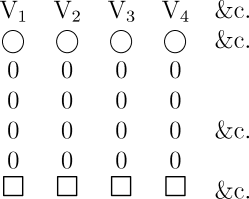The V's are for the purpose of convenient reference to any column, either in writing or speaking, and are consequently numbered. The reason why the letter V is chosen for the purpose in preference to any other letter, is because these columns are designated (as the reader will find in proceeding with the Memoir) the Variables, and sometimes the Variable columns, or the columns of Variables. The origin of this appellation is, that the values on the columns are destined to change, that is to vary, in every conceivable manner. But it is necessary to guard against the natural misapprehension that the columns are only intended to receive the values of the variables in an analytical formula, and not of the constants. The columns are called Variables on a ground wholly unconnected with the analytical distinction between constants and variables. In order to prevent the possibility of confusion, we have, both in the translation and in the notes, written Variable with a capital letter when we use the word to signify a column of the engine, and variable with a small letter when we mean the variable of a formula. Similarly, Variable-cards signify any cards that belong to a column of the engine.

To return to the explanation of the diagram: each circle at the top is intended to contain the algebraic sign + or −, either of which can be substituted for the other, according as the number represented on the column below is positive or negative. In a similar manner any other purely symbolical results of algebraical processes might be made to appear in these circles. In Note A. the practicability of developing symbolical with no less ease than numerical results has been touched on. The zeros beneath the symbolic circles represent each of them a disc, supposed to have the digit 0 presented in front. Only four tiers of zeros have been figured in the diagram, but these may be considered as representing thirty or forty, or any number of tiers of discs that may be required. Since each disc can present any digit, and each circle any sign, the discs of every column may be so adjusted as to express any positive or negative number whatever within the limits of the machine; which limits depend on the perpendicular extent of the mechanism, that is, on the number of discs to a column.

Each of the squares below the zeros is intended for the inscription of any general symbol or combination of symbols we please; it being understood that the number represented on the column immediately above is the numerical value of that symbol, or combination of symbols. Let us, for instance, represent the three quantities a, n, x, and let us further suppose that a = 5, n = 7, x = 98. We should have—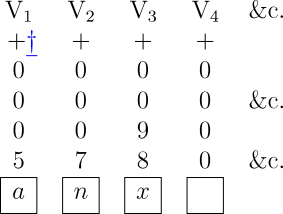Read footnote.

We may now combine these symbols in a variety of ways, so as to form any required function or functions of them, and we may then inscribe each such function below brackets, every bracket uniting together those quantities (and those only) which enter into the function inscribed below it. We must also, when we have decided on the particular function whose numerical value we desire to calculate, assign another column to the right-hand for receiving the results, and must inscribe the function in the square below this column. In the above instance we might have any one of the following functions:—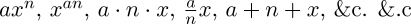Let us select the first. It would stand as follows, previous to calculation:—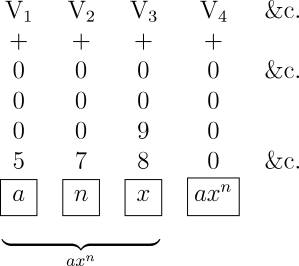The data being given, we must now put into the engine the cards proper for directing the operations in the case of the particular function chosen. These operations would in this instance be,—

First, six multiplications in order to get xn (=987 for the above particular data).

Secondly, one multiplication in order then to get a·xn (=5·987).

In all, seven multiplications to complete the whole process. We may thus represent them:—

(×, ×, ×, ×, ×, ×, ×), or 7 (×).

The multiplications would, however, at successive stages in the solution of the problem, operate on pairs of numbers, derived from different columns. In other words, the same operation would be performed on different subjects of operation. And here again is an illustration of the remarks made in the preceding Note on the independent manner in which the engine directs its operations. In determining the value of axn, the operations are homogeneous, but are distributed amongst different subjects of operation, at successive stages of the computation. It is by means of certain punched cards, belonging to the Variables themselves, that the action of the operations is so distributed as to suit each particular function. The Operation-cards merely determine the succession of operations in a general manner. They in fact throw all that portion of the mechanism included in the mill into a series of different states, which we may call the adding state, or the multiplying state, &c. respectively. In each of these states the mechanism is ready to act in the way peculiar to that state, on any pair of numbers which may be permitted to come within its sphere of action. Only one of these operating states of the mill can exist at a time; and the nature of the mechanism is also such that only one pair of numbers can be received and acted on at a time. Now, in order to secure that the mill shall receive a constant supply of the proper pairs of numbers in succession, and that it shall also rightly locate the result of an operation performed upon any pair, each Variable has cards of its own belonging to it. It has, first, a class of cards whose business it is to allow the number on the Variable to pass into the mill, there to be operated upon. These cards may be called the Supplying-cards. They furnish the mill with its proper food. Each Variable has, secondly, another class of cards, whose office it is to allow the Variable to receive a number from the mill. These cards may be called the Receiving-cards. They regulate the location of results, whether temporary or ultimate results. The Variable-cards in general (including both the preceding classes) might, it appears to us, be even more appropriately designated the Distributive-cards, since it is through their means that the action of the operations, and the results of this action, are rightly distributed.

There are two varieties of the Supplying Variable-cards, respectively adapted for fulfilling two distinct subsidiary purposes: but as these modifications do not bear upon the present subject, we shall notice them in another place.

In the above case of axn, the Operation-cards merely order seven multiplications, that is, they order the mill to be in the multiplying state seven successive times (without any reference to the particular columns whose numbers are to be acted upon). The proper Distributive Variable-cards step in at each successive multiplication, and cause the distributions requisite for the particular case.The engine might be made to calculate all these in succession. Having completed axn, the function xan might be written under the brackets instead of axn, and a new calculation commenced (the appropriate Operation and Variable-cards for the new function of course coming into play). The results would then appear on V5. So on for any number of different functions of the quantities a, n, x. Each result might either permanently remain on its column during the succeeding calculations, so that when all the functions had been computed, their values would simultaneously exist on V4, V5, V6, &c.; or each result might (after being printed off, or used in any specified manner) be effaced, to make way for its successor. The square under V4 ought, for the latter arrangement, to have the functions axn, xan, anx, &c. successively inscribed in it.

Let us now suppose that we have two expressions whose values have been computed by the engine independently of each other (each having its own group of columns for data and results). Let them be axn, and bpy. They would then stand as follows on the columns:—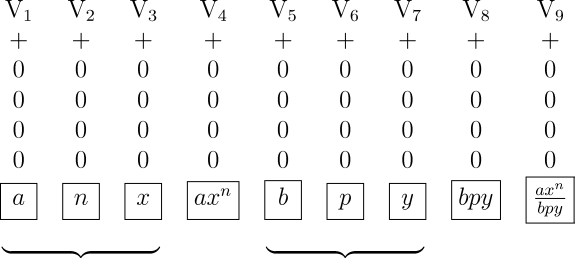We may now desire to combine together these two results, in any manner we please; in which case it would only be necessary to have an additional card or cards, which should order the requisite operations to be performed with the numbers on the two result-columns V4 and V8, and the result of these further operations to appear on a new column, V9. Say that we wish to divide axn by bpy. The numerical value of this division would then appear on the column V9, beneath which we have inscribed. The whole series of operations from the beginning would be as follows (n being = 7):

{7(×), 2(×), ÷}, or {9(×), ÷}.

This example is introduced merely to show that we may, if we please, retain separately and permanently any intermediate results (like axn, bpy) which occur in the course of processes having an ulterior and more complicated result as their chief and final object (like).

Any group of columns may be considered as representing a general function, until a special one has been implicitly impressed upon them through the introduction into the engine of the Operation and Variable-cards made out for a particular function. Thus, in the preceding example, V1, V2, V3, V5, V6, V7 represent the general function Φ(a, n, b, p, x, y) until the functionhas been determined on, and implicitly expressed by the placing of the right cards in the engine. The actual working of the mechanism, as regulated by these cards, then explicitly developes the value of the function. The inscription of a function under the brackets, and in the square under the result-column, in no way influences the processes or the results, and is merely a memorandum for the observer, to remind him of what is going on. It is the Operation and the Variable-cards only which in reality determine the function. Indeed it should be distinctly kept in mind, that the inscriptions within any of the squares are quite independent of the mechanism or workings of the engine, and are nothing but arbitrary memorandums placed there at pleasure to assist the spectator.

The further we analyse the manner in which such an engine performs its processes and attains its results, the more we perceive how distinctly it places in a true and just light the mutual relations and connexion of the various steps of mathematical analysis; how clearly it separates those things which are in reality distinct and independent, and unites those which are mutually dependent.

A. A. L.

### Note C

Those who may desire to study the principles of the Jacquard-loom in the most effectual manner, viz. that of practical observation, have only to step into the Adelaide Gallery or the Polytechnic Institution. In each of these valuable repositories of scientific illustration, a weaver is constantly working at a Jacquard-loom, and is ready to give any information that may be desired as to the construction and modes of acting of his apparatus. The volume on the manufacture of silk, in Lardner's Cyclopædia, contains a chapter on the Jacquard-loom, which may also be consulted with advantage.

The mode of application of the cards, as hitherto used in the art of weaving, was not found, however, to be sufficiently powerful for all the simplifications which it was desirable to attain in such varied and complicated processes as those required in order to fulfil the purposes of an Analytical Engine. A method was devised of what was technically designated backing the cards in certain groups according to certain laws. The object of this extension is to secure the possibility of bringing any particular card or set of cards into use any number of times successively in the solution of one problem. Whether this power shall be taken advantage of or not, in each particular instance, will depend on the nature of the operations which the problem under consideration may require. The process is alluded to by M. Menabrea, and it is a very important simplification. It has been proposed to use it for the reciprocal benefit of that art, which, while it has itself no apparent connexion with the domains of abstract science, has yet proved so valuable to the latter, in suggesting the principles which, in their new and singular field of application, seem likely to place algebraical combinations not less completely within the province of mechanism, than are all those varied intricacies of which intersecting threads are susceptible. By the introduction of the system of backing into the Jacquard-loom itself, patterns which should possess symmetry, and follow regular laws of any extent, might be woven by means of comparatively few cards.

Those who understand the mechanism of this loom will perceive that the above improvement is easily effected in practice, by causing the prism over which the train of pattern-cards is suspended to revolve backwards instead of forwards, at pleasure, under the requisite circumstances; until, by so doing, any particular card, or set of cards, that has done duty once, and passed on in the ordinary regular succession, is brought back to the position it occupied just before it was used the preceding time. The prism then resumes its forward rotation, and thus brings the card or set of cards in question into play a second time. This process may obviously be repeated any number of times.

A. A. L.

### Note D

We have represented the solution of these two equations below, with every detail, in a diagram similar to those used in Note B; but additional explanations are requisite, partly in order to make this more complicated case perfectly clear, and partly for the comprehension of certain indications and notations not used in the preceding diagrams. Those who may wish to understand Note G completely, are recommended to pay particular attention to the contents of the present Note, or they will not otherwise comprehend the similar notation and indications when applied to a much more complicated case.Clicking on the diagram displays a larger image of it. Your browser's “Back” button will return you to this document. A ready to print PostScript file for this diagram is also available for downloading in a ZIP compressed archive.

In all calculations, the columns of Variables used may be divided into three classes:—

1st. Those on which the data are inscribed:
2ndly. Those intended to receive the final results:
3rdly. Those intended to receive such intermediate and temporary combinations of the primitive data as are not to be permanently retained, but are merely needed for working with, in order to attain the ultimate results. Combinations of this kind might properly be called secondary data. They are in fact so many successive stages towards the final result. The columns which receive them are rightly named Working-Variables, for their office is in its nature purely subsidiary to other purposes. They develope an intermediate and transient class of results, which unite the original data with the final results.

The Result-Variables sometimes partake of the nature of Working-Variables. It frequently happens that a Variable destined to receive a final result is the recipient of one or more intermediate values successively, in the course of the processes. Similarly, the Variables for data often become Working-Variables, or Result-Variables, or even both in succession. It so happens, however, that in the case of the present equations the three sets of offices remain throughout perfectly separate and independent.

It will be observed, that in the squares below the Working-Variables nothing is inscribed. Any one of these Variables is in many cases destined to pass through various values successively during the performance of a calculation (although in these particular equations no instance of this occurs) . Consequently no one fixed symbol, or combination of symbols, should be considered as properly belonging to a merely Working-Variable; and as a general rule their squares are left blank. Of course in this, as in all other cases where we mention a general rule, it is understood that many particular exceptions may be expedient.

In order that all the indications contained in the diagram may be completely understood, we shall now explain two or three points, not hitherto touched on. When the value on any Variable is called into use, one of two consequences may be made to result. Either the value may return to the Variable after it has been used, in which case it is ready for a second use if needed; or the Variable may be made zero. (We are of course not considering a third case, of not unfrequent occurrence, in which the same Variable is destined to receive the result of the very operation which it has just supplied with a number.) Now the ordinary rule is, that the value returns to the Variable; unless it has been foreseen that no use for that value can recur, in which case zero is substituted. At the end of a calculation, therefore, every column ought as a general rule to be zero, excepting those for results. Thus it will be seen by the diagram, that when m, the value on V0, is used for the second time by Operation 5, V0 becomes 0, since m is not again needed; that similarly, when (mn'm'n), on V12, is used for the third time by Operation 11, V12 becomes zero, since (mn'm'n) is not again needed. In order to provide for the one or the other of the courses above indicated, there are two varieties of the Supplying Variable-cards. One of these varieties has provisions which cause the number given off from any Variable to return to that Variable after doing its duty in the mill. The other variety has provisions which cause zero to be substituted on the Variable, for the number given off. These two varieties are distinguished, when needful, by the respective appellations of the Retaining Supply-cards and the Zero Supply-cards. We see that the primary office (see Note B.) of both these varieties of cards is the same; they only differ in their secondary office.

Every Variable thus has belonging to it one class of Receiving Variable-cards and two classes of Supplying Variable-cards. It is plain however that only the one or the other of these two latter classes can be used by any one Variable for one operation; never both simultaneously, their respective functions being mutually incompatible.

It should be understood that the Variable-cards are not placed in immediate contiguity with the columns. Each card is connected by means of wires with the column it is intended to act upon.

Our diagram ought in reality to be placed side by side with M. Menabrea's corresponding table, so as to be compared with it, line for line belonging to each operation. But it was unfortunately inconvenient to print them in this desirable form. The diagram is, in the main, merely another manner of indicating the various relations denoted in M. Menabrea's table. Each mode has some advantages and some disadvantages. Combined, they form a complete and accurate method of registering every step and sequence in all calculations performed by the engine.

No notice has yet been taken of the upper indices which are added to the left of each V in the diagram; an addition which we have also taken the liberty of making to the V's in M. Menabrea's tables 3 and 4, since it does not alter anything therein represented by him, but merely adds something to the previous indications of those tables. The lower indices are obviously indices of locality only, and are wholly independent of the operations performed or of the results obtained, their value continuing unchanged during the performance of calculations. The upper indices, however, are of a different nature. Their office is to indicate any alteration in the value which a Variable represents; and they are of course liable to changes during the processes of a calculation. Whenever a Variable has only zeros upon it, it is called 0V; the moment a value appears on it (whether that value be placed there arbitrarily, or appears in the natural course of a calculation), it becomes 1V. If this value gives place to another value, the Variable becomes 2V, and so forth. Whenever a value again gives place to zero, the Variable again becomes 0V, even if it have been nV the moment before. If a value then again be substituted, the Variable becomes n+1V (as it would have done if it had not passed through the intermediate 0V); &c. &c. Just before any calculation is commenced, and after the data have been given, and everything adjusted and prepared for setting the mechanism in action, the upper indices of the Variables for data are all unity, and those for the Working and Result-variables are all zero. In this state the diagram represents them.

There are several advantages in having a set of indices of this nature; but these advantages are perhaps hardly of a kind to be immediately perceived, unless by a mind somewhat accustomed to trace the successive steps by means of which the engine accomplishes its purposes. We have only space to mention in a general way, that the whole notation of the tables is made more consistent by these indices, for they are able to mark a difference in certain cases, where there would otherwise be an apparent identity confusing in its tendency. In such a case as Vn=Vp+Vn there is more clearness and more consistency with the usual laws of algebraical notation, in being able to write m+1Vn=qVp+mVn. It is also obvious that the indices furnish a powerful means of tracing back the derivation of any result; and of registering various circumstances concerning that series of successive substitutions, of which every result is in fact merely the final consequence; circumstances that may in certain cases involve relations which it is important to observe, either for purely analytical reasons, or for practically adapting the workings of the engine to their occurrence. The series of substitutions which lead to the equations of the diagram are as follow:—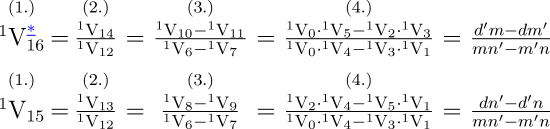Read footnote.

There are three successive substitutions for each of these equations. The formulæ (2.), (3.) and (4.) are implicitly contained in (1.), which latter we may consider as being in fact the condensed expression of any of the former. It will be observed that every succeeding substitution must contain twice as many V's as its predecessor. So that if a problem require n substitutions, the successive series of numbers for the V's in the whole of them will be 2, 4, 8, 16…2n.

The substitutions in the preceding equations happen to be of little value towards illustrating the power and uses of the upper indices, for, owing to the nature of these particular equations, the indices are all unity throughout. We wish we had space to enter more fully into the relations which these indices would in many cases enable us to trace.

M. Menabrea incloses the three centre columns of his table under the general title Variable-cards. The V's however in reality all represent the actual Variable-columns of the engine, and not the cards that belong to them. Still the title is a very just one, since it is through the special action of certain Variable-cards (when combined with the more generalized agency of the Operation-cards) that every one of the particular relations he has indicated under that title is brought about.

Suppose we wish to ascertain how often any one quantity, or combination of quantities, is brought into use during a calculation. We easily ascertain this, from the inspection of any vertical column or columns of the diagram in which that quantity may appear. Thus, in the present case, we see that all the data, and all the intermediate results likewise, are used twice, excepting (mn'm'n), which is used three times.

The order in which it is possible to perform the operations for the present example, enables us to effect all the eleven operations of which it consists with only three Operation cards; because the problem is of such a nature that it admits of each class of operations being performed in a group together; all the multiplications one after another, all the subtractions one after another, &c. The operations are {6(×), 3(-), 2(÷)}.

Since the very definition of an operation implies that there must be two numbers to act upon, there are of course two Supplying Variable-cards necessarily brought into action for every operation, in order to furnish the two proper numbers. (See Note B.) Also, since every operation must produce a result, which must be placed somewhere, each operation entails the action of a Receiving Variable-card, to indicate the proper locality for the result. Therefore, at least three times as many Variable-cards as there are operations (not Operation-cards, for these, as we have just seen, are by no means always as numerous as the operations) are brought into use in every calculation. Indeed, under certain contingencies, a still larger proportion is requisite; such, for example, would probably be the case when the same result has to appear on more than one Variable simultaneously (which is not unfrequently a provision necessary for subsequent purposes in a calculation), and in some other cases which we shall not here specify. We see therefore that a great disproportion exists between the amount of Variable and of Operation-cards requisite for the working of even the simplest calculation.

All calculations do not admit, like this one, of the operations of the same nature being performed in groups together. Probably very few do so without exceptions occurring in one or other stage of the progress; and some would not admit it at all. The order in which the operations shall be performed in every particular case is a very interesting and curious question, on which our space does not permit us fully to enter. In almost every computation a great variety of arrangements for the succession of the processes is possible, and various considerations must influence the selection amongst them for the purposes of a Calculating Engine. One essential object is to choose that arrangement which shall tend to reduce to a minimum the time necessary for completing the calculation.

It must be evident how multifarious and how mutually complicated are the considerations which the working of such an engine involve. There are frequently several distinct sets of effects going on simultaneously; all in a manner independent of each other, and yet to a greater or less degree exercising a mutual influence. To adjust each to every other, and indeed even to perceive and trace them out with perfect correctness and success, entails difficulties whose nature partakes to a certain extent of those involved in every question where conditions are very numerous and inter-complicated; such as for instance the estimation of the mutual relations amongst statistical phænomena, and of those involved in many other classes of facts.

A. A. L.

### Note E

This example has evidently been chosen on account of its brevity and simplicity, with a view merely to explain the manner in which the engine would proceed in the case of an analytical calculation containing variables, rather than to illustrate the extent of its powers to solve cases of a difficult and complex nature. The equations in first example in the Memoir are in fact a more complicated problem than the present one.

We have not subjoined any diagram of its development for this new example, as we did for the former one, because this is unnecessary after the full application already made of those diagrams to the illustration of M. Menabrea's excellent tables.

It may be remarked that a slight discrepancy exists between the formulæ

(a + bx1)
(A + B cos1 x)

given in the Memoir as the data for calculation, and the results of the calculation as developed in the last division of the table which accompanies it. To agree perfectly with this latter, the data should have been given as

(ax0 + bx1)
(A cos0 x + B cos1 x)

The following is a more complicated example of the manner in which the engine would compute a trigonometrical function containing variables. To multiply

 A+A1cos θ + A2cos 2θ + A3cos 3θ + ··· by B + B1cos θ.

Let the resulting products be represented under the general form

 C0 + C1cos θ + C2cos 2θ + C3cos 3θ + ··· (1.)

This trigonometrical series is not only in itself very appropriate for illustrating the processes of the engine, but is likewise of much practical interest from its frequent use in astronomical computations. Before proceeding further with it, we shall point out that there are three very distinct classes of ways in which it may be desired to deduce numerical values from any analytical formula.

First. We may wish to find the collective numerical value of the whole formula, without any reference to the quantities of which that formula is a function, or to the particular mode of their combination and distribution, of which the formula is the result and representative. Values of this kind are of a strictly arithmetical nature in the most limited sense of the term, and retain no trace whatever of the processes through which they have been deduced. In fact, any one such numerical value may have been attained from an infinite variety of data, or of problems. The values for x and y in the two equations (see Note D.) come under this class of numerical results.

Secondly. We may propose to compute the collective numerical value of each term of a formula, or of a series, and to keep these results separate. The engine must in such a case appropriate as many columns to results as there are terms to compute.

Thirdly. It may be desired to compute the numerical value of various subdivisions of each term, and to keep all these results separate. It may be required, for instance, to compute each coefficient separately from its variable, in which particular case the engine must appropriate two result-columns to every term that contains both a variable and coefficient.

There are many ways in which it may be desired in special cases to distribute and keep separate the numerical values of different parts of an algebraical formula; and the power of effecting such distributions to any extent is essential to the algebraical character of the Analytical Engine. Many persons who are not conversant with mathematical studies, imagine that because the business of the engine is to give its results in numerical notation, the nature of its processes must consequently be arithmetical and numerical, rather than algebraical and analytical. This is an error. The engine can arrange and combine its numerical quantities exactly as if they were letters or any other general symbols; and in fact it might bring out its results in algebraical notation, were provisions made accordingly. It might develope three sets of results simultaneously, viz. symbolic results (as already alluded to in Notes A. and B.), numerical results (its chief and primary object); and algebraical results in literal notation. This latter however has not been deemed a necessary or desirable addition to its powers, partly because the necessary arrangements for effecting it would increase the complexity and extent of the mechanism to a degree that would not be commensurate with the advantages, where the main object of the invention is to translate into numerical language general formulæ of analysis already known to us, or whose laws of formation are known to us. But it would be a mistake to suppose that because its results are given in the notation of a more restricted science, its processes are therefore restricted to those of that science. The object of the engine is in fact to give the utmost practical efficiency to the resources of numerical interpretations of the higher science of analysis, while it uses the processes and combinations of this latter.

To return to the trigonometrical series. We shall only consider the first four terms of the factor (A + A1 cos θ + &c.), since this will be sufficient to show the method. We propose to obtain separately the numerical value of each coefficient C0, C1, &c. of (1.). The direct multiplication of the two factors gives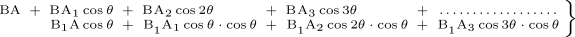(2.)

a result which would stand thus on the engine:—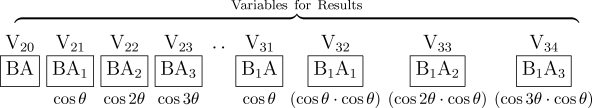The variable belonging to each coefficient is written below it, as we have done in the diagram, by way of memorandum. The only further reduction which is at first apparently possible in the preceding result, would be the addition of V21 to V31 (in which case B1A should be effaced from V31). The whole operations from the beginning would then be—

 First Series of Operations Second Series of Operations Third Series, which contains only one (final) operation 1V10×1V0 = 1V20 1V11×1V0 = 1V31 1V21×1V31 = 2V21, and 1V10×1V1 = 1V21 1V11×1V1 = 1V32 V31 becomes = 0. 1V10×1V2 = 1V22 1V11×1V2 = 1V33 1V10×1V3 = 1V23 1V11×1V3 = 1V34

We do not enter into the same detail of every step of the processes as in the examples of Notes D. and G., thinking it unnecessary and tedious to do so. The reader will remember the meaning and use of the upper and lower indices, &c., as before explained.

To proceed: we know that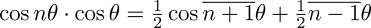(3.)

Consequently, a slight examination of the second line of (2.) will show that by making the proper substitutions, (2.) will become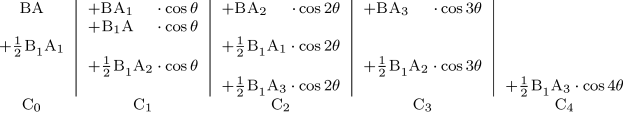These coefficients should respectively appear on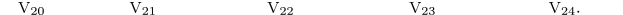We shall perceive, if we inspect the particular arrangement of the results in (2.) on the Result-columns as represented in the diagram, that, in order to effect this transformation, each successive coefficient upon V32, V33, &c. (beginning with V32), must through means of proper cards be divided by two; and that one of the halves thus obtained must be added to the coefficient on the Variable which precedes it by ten columns, and the other half to the coefficient on the Variable which precedes it by twelve columns; V32, V33, &c. themselves becoming zeros during the process.

This series of operations may be thus expressed:—

Fourth Series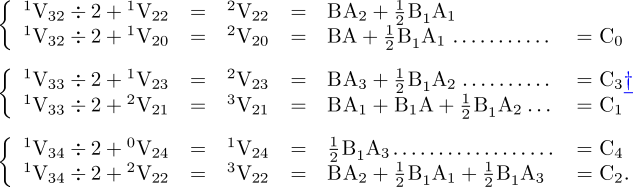Read footnote.

The calculation of the coefficients C0, C1, &c. of (1.) would now be completed, and they would stand ranged in order on V20, V21, &c. It will be remarked, that from the moment the fourth series of operations is ordered, the Variables V31, V32, &c. cease to be Result-Variables, and become mere Working-Variables.

The substitution made by the engine of the processes in the second side of (3.) for those in the first side is an excellent illustration of the manner in which we may arbitrarily order it to substitute any function, number, or process, at pleasure, for any other function, number or process, on the occurrence of a specified contingency.

We will now suppose that we desire to go a step further, and to obtain the numerical value of each complete term of the product (1.); that is, of each coefficient and variable united, which for the (n + 1)th term would be.

We must for this purpose place the variables themselves on another set of columns, V41, V42, &c., and then order their successive multiplication by V21, V22, &c., each for each. There would thus be a final series of operations as follows:—

 Fifth and Final Series of Operations 2V20 × 0V40 = 1V40 3V21 × 0V41 = 1V41 3V22 × 0V42 = 1V42 2V23 × 0V43 = 1V43 1V24 × 0V44 = 1V44

(N.B. that V40 being intended to receive the coefficient on V20 which has no variable, will only have cos 0θ (=1) inscribed on it, preparatory to commencing the fifth series of operations.)

From the moment that the fifth and final series of operations is ordered, the Variables V20, V21, &c. then in their turn cease to be Result-Variables and become mere Working-Variables; V40, V41, &c. being now the recipients of the ultimate results.

We should observe, that if the variables cos θ, cos 2θ, cos 3θ, &c. are furnished, they would be placed directly upon V41, V42, &c., like any other data. If not, a separate computation might be entered upon in a separate part of the engine, in order to calculate them, and place them on V41, &c.

We have now explained how the engine might compute (1.) in the most direct manner, supposing we knew nothing about the general term of the resulting series. But the engine would in reality set to work very differently, whenever (as in this case) we do know the law for the general term.

The first two terms of (1.) are(4.)

and the general term for all after these is(5.)

which is the coefficient of the (n+1)th term. The engine would calculate the first two terms by means of a separate set of suitable Operation-cards, and would then need another set for the third term; which last set of Operation-cards would calculate all the succeeding terms ad infinitum, merely requiring certain new Variable-cards for each term to direct the operations to act on the proper columns. The following would be the successive sets of operations for computing the coefficients of n+2 terms:—

(×, ×, ÷, +), (×, ×, ×, ÷, +, +), n(×, +, ×, ÷, +).

Or we might represent them as follows, according to the numerical order of the operations:—

(1, 2…4), (5, 6…10), n(11, 12…15).

The brackets, it should be understood, point out the relation in which the operations may be grouped, while the comma marks succession. The symbol + might be used for this latter purpose, but this would be liable to produce confusion, as + is also necessarily used to represent one class of the actual operations which are the subject of that succession. In accordance with this meaning attached to the comma, care must be taken when any one group of operations recurs more than once, as is represented above by n(11…l5), not to insert a comma after the number or letter prefixed to that group. n, (11…15) would stand for an operation n, followed by the group of operations (11…15); instead of denoting the number of groups which are to follow each other.

Wherever a general term exists, there will be a recurring group of operations, as in the above example. Both for brevity and for distinctness, a recurring group is called a cycle. A cycle of operations, then, must be understood to signify any set of operations which is repeated more than once. It is equally a cycle, whether it be repeated twice only, or an indefinite number of times; for it is the fact of a repetition occurring at all that constitutes it such. In many cases of analysis there is a recurring group of one or more cycles; that is, a cycle of a cycle, or a cycle of cycles. For instance: suppose we wish to divide a series by a series,

 (1.)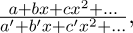it being required that the result shall be developed, like the dividend and the divisor, in successive powers of x. A little consideration of (1.), and of the steps through which algebraical division is effected, will show that (if the denominator be supposed to consist of p terms) the first partial quotient will be completed by the following operations:—

 (2.) {(÷), p(×, −)}   or   {(1), p(2, 3)},

that the second partial quotient will be completed by an exactly similar set of operations, which acts on the remainder obtained by the first set, instead of on the original dividend. The whole of the processes therefore that have been gone through, by the time the second partial quotient has been obtained, will be,—

 (3.) 2{(÷), p( × , −)}   or   2{(1), p(2, 3)},

which is a cycle that includes a cycle, or a cycle of the second order. The operations for the complete division, supposing we propose to obtain n terms of the series constituting the quotient, will be,—

 (4.) n{(÷), p( × , −)}   or   n{(1), p(2, 3)},

It is of course to be remembered that the process of algebraical division in reality continues ad infinitum, except in the few exceptional cases which admit of an exact quotient being obtained. The number n in the formula (4.) is always that of the number of terms we propose to ourselves to obtain; and the nth partial quotient is the coefficient of the (n-1)th power of x.

There are some cases which entail cycles of cycles of cycles, to an indefinite extent. Such cases are usually very complicated, and they are of extreme interest when considered with reference to the engine. The algebraical development in a series of the nth function of any given function is of this nature. Let it be proposed to obtain the nth function of

 (5.) Φ(a, b, c, …, x), x being the variable.

We should premise, that we suppose the reader to understand what is meant by an nth function. We suppose him likewise to comprehend distinctly the difference between developing an nth function algebraically, and merely calculating an nth function arithmetically. If he does not, the following will be by no means very intelligible; but we have not space to give any preliminary explanations. To proceed: the law, according to which the successive functions of (5.) are to be developed, must of course first be fixed on. This law may be of very various kinds. We may propose to obtain our results in successive powers of x, in which case the general form would be

C + C1x + C2x2 + &c.;

or in successive powers of n itself, the index of the function we are ultimately to obtain, in which case the general form would be

C + C1n + C2n2 + &c.;

and x would only enter in the coefficients. Again, other functions of x or of n instead of powers might be selected. It might be in addition proposed, that the coefficients themselves should be arranged according to given functions of a certain quantity. Another mode would be to make equations arbitrarily amongst the coefficients only, in which case the several functions, according to either of which it might be possible to develope the nth function of (5.), would have to be determined from the combined consideration of these equations and of (5.) itself.

The algebraical nature of the engine (so strongly insisted on in a previous part of this Note) would enable it to follow out any of these various modes indifferently; just as we recently showed that it can distribute and separate the numerical results of any one prescribed series of processes, in a perfectly arbitrary manner. Were it otherwise, the engine could merely compute the arithmetical nth function, a result which, like any other purely arithmetical results, would be simply a collective number, bearing no traces of the data or the processes which had led to it.

Secondly, the law of development for the nth function being selected, the next step would obviously be to develope (5.) itself, according to this law. This result would be the first function, and would be obtained by a determinate series of processes. These in most cases would include amongst them one or more cycles of operations.

The third step (which would consist of the various processes necessary for effecting the actual substitution of the series constituting the first function, for the variable itself) might proceed in either of two ways. It might make the substitution either wherever x occurs in the original (5.), or it might similarly make it wherever x occurs in the first function itself which is the equivalent of (5.). In some cases the former mode might be best, and in others the latter.

Whichever is adopted, it must be understood that the result is to appear arranged in a series following the law originally prescribed for the development of the nth function. This result constitutes the second function; with which we are to proceed exactly as we did with the first function, in order to obtain the third function, and so on, n-1 times, to obtain the nth function. We easily perceive that since every successive function is arranged in a series following the same law, there would (after the first function is obtained) be a cycle of a cycle of a cycle, &c. of operations, one, two, three, up to n-1 times, in order to get the nth function. We say, after the first function is obtained, because (for reasons on which we cannot here enter) the first function might in many cases be developed through a set of processes peculiar to itself, and not recurring for the remaining functions.

We have given but a very slight sketch of the principal general steps which would be requisite for obtaining an nth function of such a formula as (5.). The question is so exceedingly complicated, that perhaps few persons can be expected to follow, to their own satisfaction, so brief and general a statement as we are here restricted to on this subject. Still it is a very important case as regards the engine, and suggests ideas peculiar to itself, which we should regret to pass wholly without allusion. Nothing could be more interesting than to follow out, in every detail, the solution by the engine of such a case as the above; but the time, space and labour this would necessitate, could only suit a very extensive work.

To return to the subject of cycles of operations: some of the notation of the integral calculus lends itself very aptly to express them: (2.) might be thus written:—

 (6.)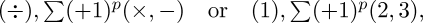where p stands for the variable; (+ 1)p for the function of the variable, that is, for Φp; and the limits are from 1 to p, or from 0 to p-1, each increment being equal to unity. Similarly, (4.) would be,—

 (7.)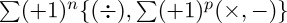the limits of n being from 1 to n, or from 0 to n-1,

 (8.) or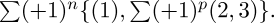Perhaps it may be thought that this notation is merely a circuitous way of expressing what was more simply and as effectually expressed before; and, in the above example, there may be some truth in this. But there is another description of cycles which can only effectually be expressed, in a condensed form, by the preceding notation. We shall call them varying cycles. They are of frequent occurrence, and include successive cycles of operations of the following nature:—

 (9.)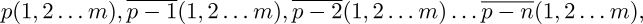where each cycle contains the same group of operations, but in which the number of repetitions of the group varies according to a fixed rate, with every cycle. (9.) can be well expressed as follows:—

 (10.), the limits of p being from p-n to p.

Independent of the intrinsic advantages which we thus perceive to result in certain cases from this use of the notation of the integral calculus, there are likewise considerations which make it interesting, from the connections and relations involved in this new application. It has been observed in some of the former Notes, that the processes used in analysis form a logical system of much higher generality than the applications to number merely. Thus, when we read over any algebraical formula, considering it exclusively with reference to the processes of the engine, and putting aside for the moment its abstract signification as to the relations of quantity, the symbols +, ×, &c. in reality represent (as their immediate and proximate effect, when the formula is applied to the engine) that a certain prism which is a part of the mechanism (see Note C.) turns a new face, and thus presents a new card to act on the bundles of levers of the engine; the new card being perforated with holes, which are arranged according to the peculiarities of the operation of addition, or of multiplication, &c. Again, the numbers in the preceding formula (8.), each of them really represents one of these very pieces of card that are hung over the prism.

Now in the use made in the formulæ (7.), (8.) and (10.), of the notation of the integral calculus, we have glimpses of a similar new application of the language of the higher mathematics. Σ, in reality, here indicates that when a certain number of cards have acted in succession, the prism over which they revolve must rotate backwards, so as to bring those cards into their former position; and the limits 1 to n, 1 to p, &c., regulate how often this backward rotation is to be repeated.

A. A. L.

### Note F

There is in existence a beautiful woven portrait of Jacquard, in the fabrication of which 24,000 cards were required.

The power of repeating the cards, alluded to by M. Menabrea, and more fully explained in Note C., reduces to an immense extent the number of cards required. It is obvious that this mechanical improvement is especially applicable wherever cycles occur in the mathematical operations, and that, in preparing data for calculations by the engine, it is desirable to arrange the order and combination of the processes with a view to obtain them as much as possible symmetrically and in cycles, in order that the mechanical advantages of the backing system may be applied to the utmost. It is here interesting to observe the manner in which the value of an analytical resource is met and enhanced by an ingenious mechanical contrivance. We see in it an instance of one of those mutual adjustments between the purely mathematical and the mechanical departments, mentioned in Note A. as being a main and essential condition of success in the invention of a calculating engine. The nature of the resources afforded by such adjustments would be of two principal kinds. In some cases, a difficulty (perhaps in itself insurmountable) in the one department would be overcome by facilities in the other; and sometimes (as in the present case) a strong point in the one would be rendered still stronger and more available by combination with a corresponding strong point in the other.

As a mere example of the degree to which the combined systems of cycles and of backing can diminish the number of cards requisite, we shall choose a case which places it in strong evidence, and which has likewise the advantage of being a perfectly different kind of problem from those that are mentioned in any of the other Notes. Suppose it be required to eliminate nine variables from ten simple equations of the form—

 ax0 + bx1 + cx2 + dx3 + ··· = p (1.) a1x0 + b1x1 + c1x2 + d1x3 + ··· = p' (2.) &c.        &c.      &c. &c.

We should explain, before proceeding, that it is not our object to consider this problem with reference to the actual arrangement of the data on the Variables of the engine, but simply as an abstract question of the nature and number of the operations required to be performed during its complete solution.

The first step would be the elimination of the first unknown quantity x0 between the first two equations. This would be obtained by the form—

(a1a-aa1)x0 + (a1b-ab1)x1 + (a1c-ac1)x2 +
+ (a1d-ad1)x3 + · · · · · · · · · · · · · · · · · · · · · · · · = a1p-ap1,

for which the operations 10 (×, ×, −) would be needed. The second step would be the elimination of x0 between the second and third equations, for which the operations would be precisely the same. We should then have had altogether the following operations:—

10(×, ×, −), 10(×, ×, −) = 20(×, ×, −)

Continuing in the same manner, the total number of operations for the complete elimination of x0 between all the successive pairs of equations would be—

9 · 10(×, ×, −) = 90(×, ×, −)

We should then be left with nine simple equations of nine variables from which to eliminate the next variable x1, for which the total of the processes would be

8 · 9(×, ×, −) = 72(×, ×, −)

We should then be left with eight simple equations of eight variables from which to eliminate x2, for which the processes would be—

7 · 8(×, ×, −) = 56(×, ×, −)

and so on. The total operations for the elimination of all the variables would thus be—

9·10 + 8·9 + 7·8 + 6·7 + 5·6 + 4·5 + 3·4 + 2·3 + 1·2 = 330.

So that three Operation-cards would perform the office of 330 such cards.

If we take n simple equations containing n−1 variables, n being a number unlimited in magnitude, the case becomes still more obvious, as the same three cards might then take the place of thousands or millions of cards.

We shall now draw further attention to the fact, already noticed, of its being by no means necessary that a formula proposed for solution should ever have been actually worked out, as a condition for enabling the engine to solve it. Provided we know the series of operations to be gone through, that is sufficient. In the foregoing instance this will be obvious enough on a slight consideration. And it is a circumstance which deserves particular notice, since herein may reside a latent value of such an engine almost incalculable in its possible ultimate results. We already know that there are functions whose numerical value it is of importance for the purposes both of abstract and of practical science to ascertain, but whose determination requires processes so lengthy and so complicated, that, although it is possible to arrive at them through great expenditure of time, labour and money, it is yet on these accounts practically almost unattainable; and we can conceive there being some results which it may be absolutely impossible in practice to attain with any accuracy, and whose precise determination it may prove highly important for some of the future wants of science, in its manifold, complicated and rapidly-developing fields of inquiry, to arrive at.

Without, however, stepping into the region of conjecture, we will mention a particular problem which occurs to us at this moment as being an apt illustration of the use to which such an engine may be turned for determining that which human brains find it difficult or impossible to work out unerringly. In the solution of the famous problem of the Three Bodies, there are, out of about 295 coefficients of lunar perturbations given by M. Clausen (Astroe. Nachrichten, No. 406) as the result of the calculations by Burg, of two by Damoiseau, and of one by Burckhardt, fourteen coefficients that differ in the nature of their algebraic sign; and out of the remainder there are only 101 (or about one-third) that agree precisely both in signs and in amount. These discordances, which are generally small in individual magnitude, may arise either from an erroneous determination of the abstract coefficients in the development of the problem, or from discrepancies in the data deduced from observation, or from both causes combined. The former is the most ordinary source of error in astronomical computations, and this the engine would entirely obviate.

We might even invent laws for series or formulæ in an arbitrary manner, and set the engine to work upon them, and thus deduce numerical results which we might not otherwise have thought of obtaining; but this would hardly perhaps in any instance be productive of any great practical utility, or calculated to rank higher than as a philosophical amusement.

A. A. L.

### Note G

It is desirable to guard against the possibility of exaggerated ideas that might arise as to the powers of the Analytical Engine. In considering any new subject, there is frequently a tendency, first, to overrate what we find to be already interesting or remarkable; and, secondly, by a sort of natural reaction, to undervalue the true state of the case, when we do discover that our notions have surpassed those that were really tenable.

The Analytical Engine has no pretensions whatever to originate anything. It can do whatever we know how to order it to perform. It can follow analysis; but it has no power of anticipating any analytical relations or truths. Its province is to assist us in making available what we are already acquainted with. This it is calculated to effect primarily and chiefly of course, through its executive faculties; but it is likely to exert an indirect and reciprocal influence on science itself in another manner. For, in so distributing and combining the truths and the formulæ of analysis, that they may become most easily and rapidly amenable to the mechanical combinations of the engine, the relations and the nature of many subjects in that science are necessarily thrown into new lights, and more profoundly investigated. This is a decidedly indirect, and a somewhat speculative, consequence of such an invention. It is however pretty evident, on general principles, that in devising for mathematical truths a new form in which to record and throw themselves out for actual use, views are likely to be induced, which should again react on the more theoretical phase of the subject. There are in all extensions of human power, or additions to human knowledge, various collateral influences, besides the main and primary object attained.

To return to the executive faculties of this engine: the question must arise in every mind, are they really even able to follow analysis in its whole extent? No reply, entirely satisfactory to all minds, can be given to this query, excepting the actual existence of the engine, and actual experience of its practical results. We will however sum up for each reader's consideration the chief elements with which the engine works:—

1. It performs the four operations of simple arithmetic upon any numbers whatever.
2. By means of certain artifices and arrangements (upon which we cannot enter within the restricted space which such a publication as the present may admit of), there is no limit either to the magnitude of the numbers used, or to the number of quantities (either variables or constants) that may be employed.
3. It can combine these numbers and these quantities either algebraically or arithmetically, in relations unlimited as to variety, extent, or complexity.
4. It uses algebraic signs according to their proper laws, and developes the logical consequences of these laws.
5. It can arbitrarily substitute any formula for any other; effacing the first from the columns on which it is represented, and making the second appear in its stead.
6. It can provide for singular values. Its power of doing this is referred to in M. Menabrea's memoir, where he mentions the passage of values through zero and infinity. The practicability of causing it arbitrarily to change its processes at any moment, on the occurrence of any specified contingency (of which its substitution of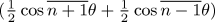for, explained in Note E., is in some degree an illustration), at once secures this point.

The subject of integration and of differentiation demands some notice. The engine can effect these processes in either of two ways:—

First. We may order it, by means of the Operation and of the Variable-cards, to go through the various steps by which the required limit can be worked out for whatever function is under consideration.

Secondly. It may (if we know the form of the limit for the function in question) effect the integration or differentiation by direct substitution. We remarked in Note B., that any set of columns on which numbers are inscribed, represents merely a general function of the several quantities, until the special function have been impressed by means of the Operation and Variable-cards. Consequently, if instead of requiring the value of the function, we require that of its integral, or of its differential coefficient, we have merely to order whatever particular combination of the ingredient quantities may constitute that integral or that coefficient. In axn, for instance, instead of the quantities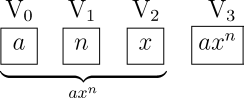being ordered to appear on V3 in the combination axn, they would be ordered to appear in that of

anxn-1

They would then stand thus:—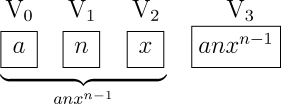Similarly, we might have, the integral of axn.

An interesting example for following out the processes of the engine would be such a form as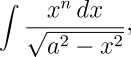or any other cases of integration by successive reductions, where an integral which contains an operation repeated n times can be made to depend upon another which contains the same n-1 or n-2 times, and so on until by continued reduction we arrive at a certain ultimate form, whose value has then to be determined.

The methods in Arbogast's Calcul des Dérivations are peculiarly fitted for the notation and the processes of the engine. Likewise the whole of the Combinatorial Analysis, which consists first in a purely numerical calculation of indices, and secondly in the distribution and combination of the quantities according to laws prescribed by these indices.

We will terminate these Notes by following up in detail the steps through which the engine could compute the Numbers of Bernoulli, this being (in the form in which we shall deduce it) a rather complicated example of its powers. The simplest manner of computing these numbers would be from the direct expansion of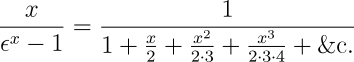(1.)

which is in fact a particular case of the development ofmentioned in Note E. Or again, we might compute them from the well-known form(2.)

or from the form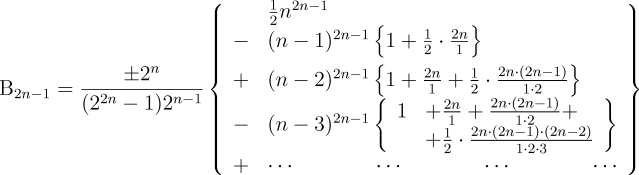(3.)

or from many others. As however our object is not simplicity or facility of computation, but the illustration of the powers of the engine, we prefer selecting the formula below, marked (8.) This is derived in the following manner:—

If in the equation(4.)

(in which B1, B3…, &c. are the Numbers of Bernoulli), we expand the denominator of the first side in powers of x, and then divide both numerator and denominator by x, we shall derive(5.)

If this latter multiplication be actually performed, we shall have a series of the general form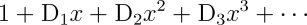(6.)

in which we see, first, that all the coefficients of the powers of x are severally equal to zero; and secondly, that the general form for D2n, the coefficient of the 2n+1th term (that is of x2n any even power of x), is the following:—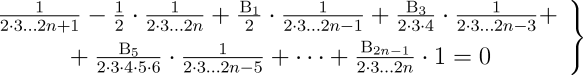(7.)

Multiplying every term by (2·3…2n) we have(8.)

which it may be convenient to write under the general form:—(9.)

A1, A3, &c. being those functions of n which respectively belong to B1, B3, &c.

We might have derived a form nearly similar to (8.), from D2n-1 the coefficient of any odd power of x in (6.); but the general form is a little different for the coefficients of the odd powers, and not quite so convenient.

On examining (7.) and (8.), we perceive that, when these formulæ are isolated from (6.), whence they are derived, and considered in themselves separately and independently, n may be any whole number whatever; although when (7.) occurs as one of the D's in (6.), it is obvious that n is then not arbitrary, but is always a certain function of the distance of that D from the beginning. If that distance be =d, thenIt is with the independent formula (8.) that we have to do. Therefore it must be remembered that the conditions for the value of n are now modified, and that n is a perfectly arbitrary whole number. This circumstance, combined with the fact (which we may easily perceive) that whatever n is, every term of (8.) after the (n+1)th is =0, and that the (n+1)th term itself is always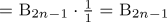, enables us to find the value (either numerical or algebraical) of any nth Number of Bernoulli B2n-1, in terms of all the preceding ones, if we but know the values of B1, B3…B2n-3. We append to this Note a Diagram and Table, containing the details of the computation for B7 (B1, B3, B5 being supposed given).

On attentively considering (8.), we shall likewise perceive that we may derive from it the numerical value of every Number of Bernoulli in succession, from the very beginning, ad infinitum, by the following series of computations:—

1st Series.—Let n=1, and calculate (8.) for this value of n. The result is B1.
2nd Series.—Let n=2. Calculate (8.) for this value of n, substituting the value of B1 just obtained. The result is B3.
3rd Series.—Let n=3. Calculate (8.) for this value of n, substituting the values of B1, B3 before obtained. The result is B5. And so on, to any extent.The diagram of the computation of the Numbers of Bernoulli is very large and intricate, and cannot be displayed as an in-line image in this document. If reduced to fit on a typical computer screen, the text in the diagram is illegible. The diagram is available at two different resolutions; the following links will display the version you select in a separate browser window (assuming your browser provides this feature), which will permit you to refer to the diagram, scrolling as necessary, while reading the following text. If you have a PostScript printer, you can download a ready-to-print PostScript file (in a ZIPped archive), which prints the diagram on a single page of paper. View diagram in new browser window: Large image (1560×1008 pixels, 69K) Monster image (1949×1260 pixels, 100K)

The diagram represents the columns of the engine when just prepared for computing B2n-1 (in the case of n=4); while the table beneath them presents a complete simultaneous view of all the successive changes which these columns then severally pass through in order to perform the computation. (The reader is referred to Note D. for explanations respecting the nature and notation of such tables.)

Six numerical data are in this case necessary for making the requisite combinations. These data are 1, 2, n(=4), B1, B3, B5. Were n=5, the additional datum B7 would be needed. Were n=6, the datum B9 would be needed; and so on. Thus the actual number of data needed will always be n+2, for n=n; and out of these n+2 data,of them are successive Numbers of Bernoulli. The reason why the Bernoulli Numbers used as data are nevertheless placed on Result-columns in the diagram, is because they may properly be supposed to have been previously computed in succession by the engine itself; under which circumstances each B will appear as a result, previous to being used as a datum for computing the succeeding B. Here then is an instance (of the kind alluded to in Note D.) of the same Variables filling more than one office in turn. It is true that if we consider our computation of B7 as a perfectly isolated calculation, we may conclude B1, B3, B5 to have been arbitrarily placed on the columns; and it would then perhaps be more consistent to put them on V4, V5, V6 as data and not results. But we are not taking this view. On the contrary, we suppose the engine to be in the course of computing the Numbers to an indefinite extent, from the very beginning; and that we merely single out, by way of example, one amongst the successive but distinct series of computations it is thus performing. Where the B's are fractional, it must be understood that they are computed and appear in the notation of decimal fractions. Indeed this is a circumstance that should be noticed with reference to all calculations. In any of the examples already given in the translation and in the Notes, some of the data, or of the temporary or permanent results, might be fractional, quite as probably as whole numbers. But the arrangements are so made, that the nature of the processes would be the same as for whole numbers.

In the above table and diagram we are not considering the signs of any of the B's, merely their numerical magnitude. The engine would bring out the sign for each of them correctly of course, but we cannot enter on every additional detail of this kind as we might wish to do. The circles for the signs are therefore intentionally left blank in the diagram.

Operation-cards 1, 2, 3, 4, 5, 6 prepare. Thus, Card 1 multiplies two into n, and the three Receiving Variable-cards belonging respectively to V4, V5, V6, allow the result 2n to be placed on each of these latter columns (this being a case in which a triple receipt of the result is needed for subsequent purposes); we see that the upper indices of the two Variables used, during Operation 1, remain unaltered.

We shall not go through the details of every operation singly, since the table and diagram sufficiently indicate them; we shall merely notice some few peculiar cases.

By Operation 6, a positive quantity is turned into a negative quantity, by simply subtracting the quantity from a column which has only zero upon it. (The sign at the top of V8 would become − during this process.)

Operation 7 will be unintelligible, unless it be remembered that if we were calculating for n = 1 instead of n = 4, Operation 6 would have completed the computation of B1 itself, in which case the engine instead of continuing its processes, would have to put B1 on V21; and then either to stop altogether, or to begin Operations 1, 2…7 all over again for value of n(=2), in order to enter on the computation of B3; (having however taken care, previous to this recommencement, to make the number on V3 equal to two, by the addition of unity to the former n=1 on that column). Now Operation 7 must either bring out a result equal to zero (if n=1); or a result greater than zero, as in the present case; and the engine follows the one or the other of the two courses just explained, contingently on the one or the other result of Operation 7. In order fully to perceive the necessity of this experimental operation, it is important to keep in mind what was pointed out, that we are not treating a perfectly isolated and independent computation, but one out of a series of antecedent and prospective computations.

Cards 8, 9, 10 produce. In Operation 9 we see an example of an upper index which again becomes a value after having passed from preceding values to zero. V11 has successively been 0V11, 1V11, 2V11, 0V11, 3V11; and, from the nature of the office which V11 performs in the calculation, its index will continue to go through further changes of the same description, which, if examined, will be found to be regular and periodic.

Card 12 has to perform the same office as Card 7 did in the preceding section; since, if n had been =2, the 11th operation would have completed the computation of B3.

Cards 13 to 20 make A3. Since A2n-1 always consists of 2n-1 factors, A3 has three factors; and it will be seen that Cards 13, 14, 15, 16 make the second of these factors, and then multiply it with the first; and that 17, 18, 19, 20 make the third factor, and then multiply this with the product of the two former factors.

Card 23 has the office of Cards 11 and 7 to perform, since if n were =3, the 21st and 22nd operations would complete the computation of B5. As our case is B7, the computation will continue one more stage; and we must now direct attention to the fact, that in order to compute A7 it is merely necessary precisely to repeat the group of Operations 13 to 20; and then, in order to complete the computation of B7, to repeat Operations 21, 22.

It will be perceived that every unit added to n in B2n-1, entails an additional repetition of operations (13…23) for the computation of B2n-1. Not only are all the operations precisely the same however for every such repetition, but they require to be respectively supplied with numbers from the very same pairs of columns; with only the one exception of Operation 21, which will of course need B5 (from V23) instead of B3 (from V22). This identity in the columns which supply the requisite numbers must not be confounded with identity in the values those columns have upon them and give out to the mill. Most of those values undergo alterations during a performance of the operations (13…23), and consequently the columns present a new set of values for the next performance of (13…23) to work on.

At the termination of the repetition of operations (13…23) in computing B7, the alterations in the values on the Variables are, that

 V6 = 2n-4 instead of 2n-2. V7 = 6 . . . . . . . . . . . . . 4. V10 = 0 . . . . . . . . . . . . . 1. V13 = A0+A1B1+A3B3+A5B5 instead of A0+A1B1+A3B3.

In this state the only remaining processes are, first, to transfer the value which is on V13 to V24; and secondly, to reduce V6, V7, V13 to zero, and to add one to V3, in order that the engine may be ready to commence computing B9. Operations 24 and 25 accomplish these purposes. It may be thought anomalous that Operation 25 is represented as leaving the upper index of V3 still=unity; but it must be remembered that these indices always begin anew for a separate calculation, and that Operation 25 places upon V3 the first value for the new calculation.

It should be remarked, that when the group (13…23) is repeated, changes occur in some of the upper indices during the course of the repetition: for example, 3V6 would become 4V6 and 5V6.

We thus see that when n=1, nine Operation-cards are used; that when n=2, fourteen Operation-cards are used; and that when n>2, twenty-five Operation-cards are used; but that no more are needed, however great n may be; and not only this, but that these same twenty-five cards suffice for the successive computation of all the Numbers from B1 to B2n-1 inclusive. With respect to the number of Variable-cards, it will be remembered, from the explanations in previous Notes, that an average of three such cards to each operation (not however to each Operation-card) is the estimate. According to this, the computation of B1 will require twenty-seven Variable-cards; B3 forty-two such cards; B5 seventy-five; and for every succeeding B after B5, there would be thirty-three additional Variable-cards (since each repetition of the group (13…23) adds eleven to the number of operations required for computing the previous B). But we must now explain, that whenever there is a cycle of operations, and if these merely require to be supplied with numbers from the same pairs of columns, and likewise each operation to place its result on the same column for every repetition of the whole group, the process then admits of a cycle of Variable-cards for effecting its purposes. There is obviously much more symmetry and simplicity in the arrangements, when cases do admit of repeating the Variable as well as the Operation-cards. Our present example is of this nature. The only exception to a perfect identity in all the processes and columns used, for every repetition of Operations (13…23), is, that Operation 21 always requires one of its factors from a new column, and Operation 24 always puts its result on a new column. But as these variations follow the same law at each repetition (Operation 21 always requiring its factor from a column one in advance of that which it used the previous time, and Operation 24 always putting its result on the column one in advance of that which received the previous result), they are easily provided for in arranging the recurring group (or cycle) of Variable-cards.

We may here remark, that the average estimate of three Variable-cards coming into use to each operation, is not to be taken as an absolutely and literally correct amount for all cases and circumstances. Many special circumstances, either in the nature of a problem, or in the arrangements of the engine under certain contingencies, influence and modify this average to a greater or less extent; but it is a very safe and correct general rule to go upon. In the preceding case it will give us seventy-five Variable-cards as the total number which will be necessary for computing any B after B3. This is very nearly the precise amount really used, but we cannot here enter into the minutiæ of the few particular circumstances which occur in this example (as indeed at some one stage or other of probably most computations) to modify slightly this number.

It will be obvious that the very same seventy-five Variable-cards may be repeated for the computation of every succeeding Number, just on the same principle as admits of the repetition of the thirty-three Variable-cards of Operations (13…23) in the computation of any one Number. Thus there will be a cycle of a cycle of Variable-cards.

If we now apply the notation for cycles, as explained in Note E., we may express the operations for computing the Numbers of Bernoulli in the following manner:—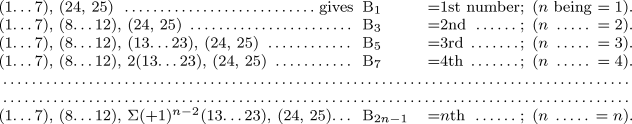Again,represents the total operations for computing every number in succession, from B1 to B2n-1 inclusive.

In this formula we see a varying cycle of the first order, and an ordinary cycle of the second order. The latter cycle in this case includes in it the varying cycle.

On inspecting the ten Working-Variables of the diagram, it will be perceived, that although the value on any one of them (excepting V4 and V5) goes through a series of changes, the office which each performs is in this calculation fixed and invariable. Thus V6 always prepares the numerators of the factors of any A; V7 the denominators. V8 always receives the (2n-3)th factor of A2n-1, and V9 the (2n-1)th. V10 always decides which of two courses the succeeding processes are to follow, by feeling for the value of n through means of a subtraction; and so on; but we shall not enumerate further. It is desirable in all calculations so to arrange the processes, that the offices performed by the Variables may be as uniform and fixed as possible.

Supposing that it was desired not only to tabulate B1, B3, &c., but A0, A1, &c.; we have only then to appoint another series of Variables, V41, V42, &c., for receiving these latter results as they are successively produced upon V11. Or again, we may, instead of this, or in addition to this second series of results, wish to tabulate the value of each successive total term of the series (8.), viz. A0, A1B1, A3B3, &c. We have then merely to multiply each B with each corresponding A, as produced, and to place these successive products on Result-columns appointed for the purpose.

The formula (8.) is interesting in another point of view. It is one particular case of the general Integral of the following Equation of Mixed Differences:—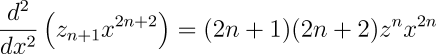for certain special suppositions respecting z, x and n.

The general integral itself is of the form,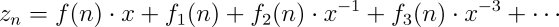and it is worthy of remark, that the engine might (in a manner more or less similar to the preceding) calculate the value of this formula upon most other hypotheses for the functions in the integral with as much, or (in many cases) with more ease than it can formula (8.).

A. A. L.